### Fiery Dragon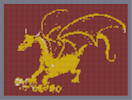Hover over the thumbnail for a full-size version.

Author kinglibai author:kinglibai dragon n-art non-playable nonplayable rated 2007-12-17 2007-12-20 3 by 10 people. \$Fiery Dragon#kinglibai#none#00000000000000000000000000000000000000000000000000000000000000000000000000000000000000000000000000000000000000000000000000000000000000000000000000000000000000000000000000000000000000000000000000000000000000000000000000000000000000000000000000000000000000000000000000000000000000000000000000000000000000000000000000000000000000000000000000000000000000000000000000000000000000000000000000000000000000000000000000000000000000000000000000000000000000000000000000000000000000000000000000000000000000000000000000000000000000000000000000000000000000000000000000000000000000000000000000000000000000000000000000000000000000000000000000000000000000000000000000000000000000000000000000000000000000000000000000000000000000000|0^780,588!12^24,24!12^24,30!12^24,36!12^24,42!12^24,48!12^24,54!12^24,60!12^24,66!12^24,72!12^24,78!12^24,84!12^24,90!12^24,96!12^24,102!12^24,108!12^24,114!12^24,120!12^24,126!12^24,132!12^24,138!12^24,144!12^24,150!12^24,156!12^24,162!12^24,168!12^24,174!12^24,180!12^24,186!12^24,192!12^24,198!12^24,204!12^24,210!12^24,216!12^24,222!12^24,228!12^24,234!12^24,240!12^24,246!12^24,252!12^24,258!12^24,264!12^24,270!12^24,276!12^24,282!12^24,288!12^24,294!12^24,300!12^24,306!12^24,312!12^24,318!12^24,324!12^24,330!12^24,336!12^24,342!12^24,348!12^24,354!12^24,360!12^24,366!12^24,372!12^24,378!12^24,384!12^24,390!12^24,396!12^24,402!12^24,408!12^24,414!12^24,420!12^24,426!12^24,432!12^24,438!12^24,444!12^24,450!12^24,456!12^24,462!12^24,468!12^24,474!12^24,480!12^24,486!12^24,492!12^24,498!12^24,504!12^24,510!12^24,516!12^24,522!12^24,528!12^24,534!12^24,540!12^24,546!12^24,552!12^24,558!12^24,564!12^24,570!12^24,576!12^30,24!12^30,30!12^30,36!12^30,42!12^30,48!12^30,54!12^30,60!12^30,66!12^30,72!12^30,78!12^30,84!12^30,90!12^30,96!12^30,102!12^30,108!12^30,114!12^30,120!12^30,126!12^30,132!12^30,138!12^30,144!12^30,150!12^30,156!12^30,162!12^30,168!12^30,174!12^30,180!12^30,186!12^30,192!12^30,198!12^30,204!12^30,210!12^30,216!12^30,222!12^30,228!12^30,234!12^30,240!12^30,246!12^30,252!12^30,258!12^30,264!12^30,270!12^30,276!12^30,282!12^30,288!12^30,294!12^30,300!12^30,306!12^30,312!12^30,318!12^30,324!12^30,330!12^30,336!12^30,342!12^30,348!12^30,354!12^30,360!12^30,366!12^30,372!12^30,378!12^30,384!12^30,390!12^30,396!12^30,402!12^30,408!12^30,414!12^30,420!12^30,426!12^30,432!12^30,438!12^30,444!12^30,450!12^30,456!12^30,462!12^30,468!12^30,474!12^30,480!12^30,486!12^30,492!12^30,498!12^30,504!12^30,510!12^30,516!12^30,522!12^30,528!12^30,534!12^30,540!12^30,546!12^30,552!12^30,558!12^30,564!12^30,570!12^30,576!12^36,24!12^36,30!12^36,36!12^36,42!12^36,48!12^36,54!12^36,60!12^36,66!12^36,72!12^36,78!12^36,84!12^36,90!12^36,96!12^36,102!12^36,108!12^36,114!12^36,120!12^36,126!12^36,132!12^36,138!12^36,144!12^36,150!12^36,156!12^36,162!12^36,168!12^36,174!12^36,180!12^36,186!12^36,192!12^36,198!12^36,204!12^36,210!12^36,216!12^36,222!12^36,228!12^36,234!12^36,240!12^36,246!12^36,252!12^36,258!12^36,264!12^36,270!12^36,276!12^36,282!12^36,288!12^36,294!12^36,300!12^36,306!12^36,312!12^36,318!12^36,324!12^36,330!12^36,336!12^36,342!12^36,348!12^36,354!12^36,360!12^36,366!12^36,372!12^36,378!12^36,384!12^36,390!12^36,396!12^36,402!12^36,408!12^36,414!12^36,420!12^36,426!12^36,432!12^36,438!12^36,444!12^36,450!12^36,456!12^36,462!12^36,468!12^36,474!12^36,480!12^36,486!12^36,492!12^36,498!12^36,504!12^36,510!12^36,516!12^36,522!12^36,528!12^36,534!12^36,540!12^36,546!12^36,552!12^36,558!12^36,564!12^36,570!12^36,576!12^42,24!12^42,30!12^42,36!12^42,42!12^42,48!12^42,54!12^42,60!12^42,66!12^42,72!12^42,78!12^42,84!12^42,90!12^42,96!12^42,102!12^42,108!12^42,114!12^42,120!12^42,126!12^42,132!12^42,138!12^42,144!12^42,150!12^42,156!12^42,162!12^42,168!12^42,174!12^42,180!12^42,186!12^42,192!12^42,198!12^42,204!12^42,210!12^42,216!12^42,222!12^42,228!12^42,234!12^42,240!12^42,246!12^42,252!12^42,258!12^42,264!12^42,270!12^42,276!12^42,282!12^42,288!12^42,294!12^42,300!12^42,306!12^42,312!12^42,318!12^42,324!12^42,330!12^42,336!12^42,342!12^42,348!12^42,354!12^42,360!12^42,366!12^42,372!12^42,378!12^42,384!12^42,390!12^42,396!12^42,402!12^42,408!12^42,414!12^42,420!12^42,426!12^42,432!12^42,438!12^42,444!12^42,450!12^42,456!12^42,462!12^42,468!12^42,474!12^42,480!12^42,486!12^42,492!12^42,498!12^42,504!12^42,510!12^42,516!12^42,522!12^42,528!12^42,534!12^42,540!12^42,546!12^42,552!12^42,558!12^42,564!12^42,570!12^42,576!12^48,24!12^48,30!12^48,36!12^48,42!12^48,48!12^48,54!12^48,60!12^48,66!12^48,72!12^48,78!12^48,84!12^48,90!12^48,96!12^48,102!12^48,108!12^48,114!12^48,120!12^48,126!12^48,132!12^48,138!12^48,144!12^48,150!12^48,156!12^48,162!12^48,168!12^48,174!12^48,180!12^48,186!12^48,192!12^48,198!12^48,204!12^48,210!12^48,216!12^48,222!12^48,228!12^48,234!12^48,240!12^48,246!12^48,252!12^48,258!12^48,264!12^48,270!12^48,276!12^48,282!12^48,288!12^48,294!12^48,300!12^48,306!12^48,312!12^48,318!12^48,324!12^48,330!12^48,336!12^48,342!12^48,348!12^48,354!12^48,360!12^48,366!12^48,372!12^48,378!12^48,384!12^48,390!12^48,396!12^48,402!12^48,408!12^48,414!12^48,420!12^48,426!12^48,432!12^48,438!12^48,444!12^48,450!12^48,456!12^48,462!12^48,468!12^48,474!12^48,480!12^48,486!12^48,492!12^48,498!12^48,504!12^48,510!12^48,516!12^48,522!12^48,528!12^48,534!12^48,540!12^48,546!12^48,552!12^48,558!12^48,564!12^48,570!12^48,576!12^54,24!12^54,30!12^54,36!12^54,42!12^54,48!12^54,54!12^54,60!12^54,66!12^54,72!12^54,78!12^54,84!12^54,90!12^54,96!12^54,102!12^54,108!12^54,114!12^54,120!12^54,126!12^54,132!12^54,138!12^54,144!12^54,150!12^54,156!12^54,162!12^54,168!12^54,174!12^54,180!12^54,186!12^54,192!12^54,198!12^54,204!12^54,210!12^54,216!12^54,222!12^54,228!12^54,234!12^54,240!12^54,246!12^54,252!12^54,258!12^54,264!12^54,270!12^54,276!12^54,282!12^54,288!12^54,294!12^54,300!12^54,306!12^54,312!12^54,318!12^54,324!12^54,330!12^54,336!12^54,342!12^54,348!12^54,354!12^54,360!12^54,366!12^54,372!12^54,378!12^54,384!12^54,390!12^54,396!12^54,402!12^54,408!12^54,414!12^54,420!12^54,426!12^54,432!12^54,438!12^54,444!12^54,450!12^54,456!12^54,462!12^54,468!12^54,474!12^54,480!12^54,486!12^54,492!12^54,498!12^54,504!12^54,510!12^54,516!12^54,522!12^54,528!12^54,534!12^54,540!12^54,546!12^54,552!12^54,558!12^54,564!12^54,570!12^54,576!12^60,24!12^60,30!12^60,36!12^60,42!12^60,48!12^60,54!12^60,60!12^60,66!12^60,72!12^60,78!12^60,84!12^60,90!12^60,96!12^60,102!12^60,108!12^60,114!12^60,120!12^60,126!12^60,132!12^60,138!12^60,144!12^60,150!12^60,156!12^60,162!12^60,168!12^60,174!12^60,180!12^60,186!12^60,192!12^60,198!12^60,204!12^60,210!12^60,216!12^60,222!12^60,228!12^60,234!12^60,240!12^60,246!12^60,252!12^60,258!12^60,264!12^60,270!12^60,276!12^60,282!12^60,288!12^60,294!12^60,300!12^60,306!12^60,312!12^60,318!12^60,324!12^60,330!12^60,336!12^60,342!12^60,348!12^60,354!12^60,360!12^60,366!12^60,372!12^60,378!12^60,384!12^60,390!12^60,396!12^60,402!12^60,408!12^60,414!12^60,420!12^60,426!12^60,432!12^60,438!12^60,444!12^60,450!12^60,456!12^60,462!12^60,468!12^60,474!12^60,480!12^60,486!12^60,492!12^60,498!12^60,504!12^60,510!12^60,516!12^60,522!12^60,528!12^60,534!12^60,540!12^60,546!12^60,552!12^60,558!12^60,564!12^60,570!12^60,576!12^66,24!12^66,30!12^66,36!12^66,42!12^66,48!12^66,54!12^66,60!12^66,66!12^66,72!12^66,78!12^66,84!12^66,90!12^66,96!12^66,102!12^66,108!12^66,114!12^66,120!12^66,126!12^66,132!12^66,138!12^66,144!12^66,150!12^66,156!12^66,162!12^66,168!12^66,174!12^66,180!12^66,186!12^66,192!12^66,198!12^66,204!12^66,210!12^66,216!12^66,222!12^66,228!12^66,234!12^66,240!12^66,246!12^66,252!12^66,258!12^66,264!12^66,270!12^66,276!12^66,282!12^66,288!12^66,294!12^66,300!12^66,306!12^66,312!12^66,318!12^66,324!12^66,330!12^66,336!12^66,342!12^66,348!12^66,354!12^66,360!12^66,366!12^66,372!12^66,378!12^66,384!12^66,390!12^66,396!12^66,402!12^66,408!12^66,414!12^66,420!12^66,426!12^66,432!12^66,438!12^66,444!12^66,450!12^66,456!12^66,462!12^66,468!12^66,474!12^66,480!12^66,486!12^66,492!12^66,498!12^66,504!12^66,510!12^66,516!12^66,522!12^66,528!12^66,534!12^66,540!12^66,546!12^66,552!12^66,558!12^66,564!12^66,570!12^66,576!12^72,24!12^72,30!12^72,36!12^72,42!12^72,48!12^72,54!12^72,60!12^72,66!12^72,72!12^72,78!12^72,84!12^72,90!12^72,96!12^72,102!12^72,108!12^72,114!12^72,120!12^72,126!12^72,132!12^72,138!12^72,144!12^72,150!12^72,156!12^72,162!12^72,168!12^72,174!12^72,180!12^72,186!12^72,192!12^72,198!12^72,204!12^72,210!12^72,216!12^72,222!12^72,228!12^72,234!12^72,240!12^72,246!12^72,252!12^72,258!12^72,264!12^72,270!12^72,276!12^72,282!12^72,288!12^72,294!12^72,300!12^72,306!12^72,312!12^72,318!12^72,324!12^72,330!12^72,336!12^72,342!12^72,348!12^72,354!12^72,360!12^72,366!12^72,372!12^72,378!12^72,384!12^72,390!12^72,396!12^72,402!12^72,408!12^72,414!12^72,420!12^72,426!12^72,432!12^72,438!12^72,444!12^72,450!12^72,456!12^72,462!12^72,468!12^72,474!12^72,480!12^72,486!12^72,492!12^72,498!12^72,504!12^72,510!12^72,516!12^72,522!12^72,528!12^72,534!12^72,540!12^72,546!12^72,552!12^72,558!12^72,564!12^72,570!12^72,576!12^78,24!12^78,30!12^78,36!12^78,42!12^78,48!12^78,54!12^78,60!12^78,66!12^78,72!12^78,78!12^78,84!12^78,90!12^78,96!12^78,102!12^78,108!12^78,114!12^78,120!12^78,126!12^78,132!12^78,138!12^78,144!12^78,150!12^78,156!12^78,162!12^78,168!12^78,174!12^78,180!12^78,186!12^78,192!12^78,198!12^78,204!12^78,210!12^78,216!12^78,222!12^78,228!12^78,234!12^78,240!12^78,246!12^78,252!12^78,258!12^78,264!12^78,270!12^78,276!12^78,282!12^78,288!12^78,294!12^78,300!12^78,306!12^78,312!12^78,318!12^78,324!12^78,330!12^78,336!12^78,342!12^78,348!12^78,354!12^78,360!12^78,366!12^78,372!12^78,378!12^78,384!12^78,390!12^78,396!12^78,402!12^78,408!12^78,414!12^78,420!12^78,426!12^78,432!12^78,438!12^78,444!12^78,450!12^78,456!12^78,462!12^78,468!12^78,474!12^78,480!12^78,486!12^78,492!12^78,498!12^78,504!12^78,510!12^78,516!12^78,522!12^78,528!12^78,534!12^78,540!12^78,546!12^78,552!12^78,558!12^78,564!12^78,570!12^78,576!12^84,24!12^84,30!12^84,36!12^84,42!12^84,48!12^84,54!12^84,60!12^84,66!12^84,72!12^84,78!12^84,84!12^84,90!12^84,96!12^84,102!12^84,108!12^84,114!12^84,120!12^84,126!12^84,132!12^84,138!12^84,144!12^84,150!12^84,156!12^84,162!12^84,168!12^84,174!12^84,180!12^84,186!12^84,192!12^84,198!12^84,204!12^84,210!12^84,216!12^84,222!12^84,228!12^84,234!12^84,240!12^84,246!12^84,252!12^84,258!12^84,264!12^84,270!12^84,276!12^84,282!12^84,288!12^84,294!12^84,300!12^84,306!12^84,312!12^84,318!12^84,324!12^84,330!12^84,336!12^84,342!12^84,348!12^84,354!12^84,360!12^84,366!12^84,372!12^84,378!12^84,384!12^84,390!12^84,396!12^84,402!12^84,408!12^84,414!12^84,420!12^84,426!12^84,432!12^84,438!12^84,444!12^84,450!12^84,456!12^84,462!12^84,468!12^84,474!12^84,480!12^84,486!12^84,492!12^84,498!12^84,504!12^84,510!12^84,516!12^84,522!12^84,528!12^84,534!12^84,540!12^84,546!12^84,552!12^84,558!12^84,564!12^84,570!12^84,576!12^90,24!12^90,30!12^90,36!12^90,42!12^90,48!12^90,54!12^90,60!12^90,66!12^90,72!12^90,78!12^90,84!12^90,90!12^90,96!12^90,102!12^90,108!12^90,114!12^90,120!12^90,126!12^90,132!12^90,138!12^90,144!12^90,150!12^90,156!12^90,162!12^90,168!12^90,174!12^90,180!12^90,186!12^90,192!12^90,198!12^90,204!12^90,210!12^90,216!12^90,222!12^90,228!12^90,234!12^90,240!12^90,246!12^90,252!12^90,258!12^90,264!12^90,270!12^90,276!12^90,282!12^90,288!12^90,294!12^90,300!12^90,306!12^90,312!12^90,318!12^90,324!12^90,330!12^90,336!12^90,342!12^90,348!12^90,354!12^90,360!12^90,366!12^90,372!12^90,378!12^90,384!12^90,390!12^90,396!12^90,402!12^90,408!12^90,414!12^90,420!12^90,426!12^90,432!12^90,438!12^90,444!12^90,450!12^90,456!12^90,462!12^90,468!12^90,474!12^90,480!0^90,486!0^90,492!0^90,498!12^90,504!12^90,510!12^90,516!12^90,522!12^90,528!12^90,534!12^90,540!12^90,546!12^90,552!12^90,558!12^90,564!12^90,570!12^90,576!12^96,24!12^96,30!12^96,36!12^96,42!12^96,48!12^96,54!12^96,60!12^96,66!12^96,72!12^96,78!12^96,84!12^96,90!12^96,96!12^96,102!12^96,108!12^96,114!12^96,120!12^96,126!12^96,132!12^96,138!12^96,144!12^96,150!12^96,156!12^96,162!12^96,168!12^96,174!12^96,180!12^96,186!12^96,192!12^96,198!12^96,204!12^96,210!12^96,216!12^96,222!12^96,228!12^96,234!12^96,240!12^96,246!12^96,252!12^96,258!12^96,264!12^96,270!12^96,276!12^96,282!12^96,288!12^96,294!12^96,300!12^96,306!12^96,312!12^96,318!12^96,324!12^96,330!12^96,336!12^96,342!12^96,348!12^96,354!12^96,360!12^96,366!12^96,372!12^96,378!12^96,384!12^96,390!12^96,396!12^96,402!12^96,408!12^96,414!12^96,420!12^96,426!12^96,432!12^96,438!12^96,444!12^96,450!12^96,456!12^96,462!12^96,468!0^96,486!12^96,498!12^96,516!12^96,522!12^96,528!12^96,534!12^96,540!12^96,546!12^96,552!12^96,558!12^96,564!12^96,570!12^96,576!12^102,24!12^102,30!12^102,36!12^102,42!12^102,48!12^102,54!12^102,60!12^102,66!12^102,72!12^102,78!12^102,84!12^102,90!12^102,96!12^102,102!12^102,108!12^102,114!12^102,120!12^102,126!12^102,132!12^102,138!12^102,144!12^102,150!12^102,156!12^102,162!12^102,168!12^102,174!12^102,180!12^102,186!12^102,192!12^102,198!12^102,204!12^102,210!12^102,216!12^102,222!12^102,228!12^102,234!12^102,240!12^102,246!12^102,252!12^102,258!12^102,264!12^102,270!12^102,276!12^102,282!12^102,288!12^102,294!12^102,300!12^102,306!12^102,312!12^102,318!12^102,324!12^102,330!12^102,336!12^102,342!12^102,348!12^102,354!12^102,360!12^102,366!12^102,372!12^102,378!12^102,384!12^102,390!12^102,396!12^102,402!12^102,408!12^102,414!12^102,420!12^102,426!12^102,432!12^102,438!12^102,444!12^102,450!12^102,456!12^102,462!0^102,468!0^102,486!0^102,492!0^102,498!12^102,516!12^102,522!12^102,528!12^102,534!12^102,540!12^102,546!12^102,552!12^102,558!12^102,564!12^102,570!12^102,576!12^108,24!12^108,30!12^108,36!12^108,42!12^108,48!12^108,54!12^108,60!12^108,66!12^108,72!12^108,78!12^108,84!12^108,90!12^108,96!12^108,102!12^108,108!12^108,114!12^108,120!12^108,126!12^108,132!12^108,138!12^108,144!12^108,150!12^108,156!12^108,162!12^108,168!12^108,174!12^108,180!12^108,186!12^108,192!12^108,198!12^108,204!12^108,210!12^108,216!12^108,222!12^108,228!12^108,234!12^108,240!12^108,246!12^108,252!12^108,258!12^108,264!12^108,270!12^108,276!12^108,282!12^108,288!12^108,294!0^108,300!12^108,306!12^108,312!12^108,318!12^108,324!12^108,330!12^108,336!12^108,342!12^108,348!12^108,354!12^108,360!12^108,366!12^108,372!12^108,378!12^108,384!12^108,390!12^108,396!12^108,402!12^108,408!12^108,414!12^108,420!12^108,426!12^108,432!12^108,438!12^108,444!12^108,450!12^108,456!12^108,462!0^108,468!0^108,486!0^108,492!0^108,498!12^108,516!12^108,522!12^108,528!12^108,534!12^108,540!12^108,546!12^108,552!12^108,558!12^108,564!12^108,570!12^108,576!12^114,24!12^114,30!12^114,36!12^114,42!12^114,48!12^114,54!12^114,60!12^114,66!12^114,72!12^114,78!12^114,84!12^114,90!12^114,96!12^114,102!12^114,108!12^114,114!12^114,120!12^114,126!12^114,132!12^114,138!12^114,144!12^114,150!12^114,156!12^114,162!12^114,168!12^114,174!12^114,180!12^114,186!12^114,192!12^114,198!12^114,204!12^114,210!12^114,216!12^114,222!12^114,228!12^114,234!12^114,240!12^114,246!12^114,252!12^114,258!12^114,264!12^114,270!0^114,276!0^114,288!0^114,294!0^114,300!12^114,306!12^114,312!12^114,318!12^114,324!12^114,330!12^114,336!12^114,342!12^114,348!12^114,354!12^114,360!12^114,366!12^114,372!12^114,378!12^114,384!12^114,390!12^114,396!12^114,402!12^114,408!12^114,414!12^114,420!12^114,426!12^114,432!12^114,438!12^114,444!12^114,450!12^114,456!12^114,462!0^114,468!0^114,474!0^114,480!0^114,486!0^114,492!0^114,498!0^114,504!0^114,510!12^114,516!12^114,522!12^114,528!12^114,534!12^114,540!12^114,546!12^114,552!12^114,558!12^114,564!12^114,570!12^114,576!12^120,24!12^120,30!12^120,36!12^120,42!12^120,48!12^120,54!12^120,60!12^120,66!12^120,72!12^120,78!12^120,84!12^120,90!12^120,96!12^120,102!12^120,108!12^120,114!12^120,120!12^120,126!12^120,132!12^120,138!12^120,144!12^120,150!12^120,156!12^120,162!12^120,168!12^120,174!12^120,180!12^120,186!12^120,192!12^120,198!12^120,204!12^120,210!12^120,216!12^120,222!12^120,228!12^120,234!12^120,240!12^120,246!12^120,252!12^120,258!12^120,264!12^120,270!0^120,276!0^120,282!0^120,288!0^120,294!0^120,300!12^120,306!12^120,312!12^120,318!12^120,324!12^120,330!12^120,336!12^120,342!12^120,348!12^120,354!12^120,360!12^120,366!12^120,372!12^120,378!12^120,384!12^120,390!12^120,396!12^120,402!12^120,408!12^120,414!12^120,420!12^120,426!12^120,432!12^120,438!12^120,444!12^120,450!12^120,456!12^120,462!12^120,468!0^120,474!12^120,504!12^120,510!12^120,516!12^120,522!0^120,528!12^120,540!12^120,546!12^120,552!12^120,558!12^120,564!12^120,570!12^120,576!12^126,24!12^126,30!12^126,36!12^126,42!12^126,48!12^126,54!12^126,60!12^126,66!12^126,72!12^126,78!12^126,84!12^126,90!12^126,96!12^126,102!12^126,108!12^126,114!12^126,120!12^126,126!12^126,132!12^126,138!12^126,144!12^126,150!12^126,156!12^126,162!12^126,168!12^126,174!12^126,180!12^126,186!12^126,192!12^126,198!12^126,204!12^126,210!12^126,216!12^126,222!12^126,228!12^126,234!12^126,240!12^126,246!12^126,252!12^126,258!0^126,264!0^126,270!0^126,276!0^126,282!0^126,288!0^126,294!0^126,300!12^126,306!12^126,312!12^126,318!12^126,324!12^126,330!12^126,336!12^126,342!12^126,348!12^126,354!12^126,360!12^126,366!12^126,372!12^126,378!12^126,384!12^126,390!12^126,396!12^126,402!12^126,408!12^126,414!12^126,420!12^126,426!12^126,432!12^126,438!12^126,444!12^126,450!12^126,456!12^126,462!12^126,468!0^126,492!12^126,504!12^126,510!12^126,516!12^126,522!0^126,528!12^126,534!12^126,540!12^126,546!12^126,552!12^126,558!12^126,564!12^126,570!12^126,576!12^132,24!12^132,30!12^132,36!12^132,42!12^132,48!12^132,54!12^132,60!12^132,66!12^132,72!12^132,78!12^132,84!12^132,90!12^132,96!12^132,102!12^132,108!12^132,114!12^132,120!12^132,126!12^132,132!12^132,138!12^132,144!12^132,150!12^132,156!12^132,162!12^132,168!12^132,174!12^132,180!12^132,186!12^132,192!12^132,198!12^132,204!12^132,210!12^132,216!12^132,222!12^132,228!12^132,234!12^132,240!12^132,246!12^132,252!12^132,258!0^132,264!0^132,270!0^132,276!0^132,282!0^132,288!0^132,294!0^132,300!12^132,306!12^132,312!12^132,318!12^132,324!12^132,330!12^132,336!12^132,342!12^132,348!12^132,354!12^132,360!12^132,366!12^132,372!12^132,378!12^132,384!12^132,390!12^132,396!12^132,402!12^132,408!12^132,414!12^132,420!12^132,426!12^132,432!12^132,438!12^132,444!12^132,450!12^132,456!12^132,462!12^132,468!0^132,474!0^132,486!0^132,498!12^132,504!12^132,510!12^132,516!12^132,522!12^132,528!12^132,534!12^132,540!12^132,546!12^132,552!12^132,558!12^132,564!12^132,570!12^132,576!12^138,24!12^138,30!12^138,36!12^138,42!12^138,48!12^138,54!12^138,60!12^138,66!12^138,72!12^138,78!12^138,84!12^138,90!12^138,96!12^138,102!12^138,108!12^138,114!12^138,120!12^138,126!12^138,132!12^138,138!12^138,144!12^138,150!12^138,156!12^138,162!12^138,168!12^138,174!12^138,180!12^138,186!12^138,192!12^138,198!12^138,204!12^138,210!12^138,216!12^138,222!12^138,228!12^138,234!12^138,240!12^138,246!12^138,252!12^138,258!0^138,264!12^138,270!0^138,276!0^138,282!0^138,288!12^138,294!12^138,306!12^138,312!12^138,318!12^138,324!12^138,330!12^138,336!12^138,342!12^138,348!12^138,354!12^138,360!12^138,366!12^138,372!12^138,378!12^138,384!12^138,390!12^138,396!12^138,402!12^138,408!12^138,414!12^138,420!12^138,426!12^138,432!12^138,438!12^138,444!12^138,450!12^138,456!12^138,462!12^138,468!12^138,474!12^138,480!0^138,486!0^138,492!0^138,504!0^138,510!0^138,522!12^138,528!0^138,534!12^138,540!12^138,546!12^138,552!12^138,558!12^138,564!12^138,570!12^138,576!12^144,24!12^144,30!12^144,36!12^144,42!12^144,48!12^144,54!12^144,60!12^144,66!12^144,72!12^144,78!12^144,84!12^144,90!12^144,96!12^144,102!12^144,108!12^144,114!12^144,120!12^144,126!12^144,132!12^144,138!12^144,144!12^144,150!12^144,156!12^144,162!12^144,168!12^144,174!12^144,180!12^144,186!12^144,192!12^144,198!12^144,204!12^144,210!12^144,216!12^144,222!12^144,228!12^144,234!12^144,240!0^144,246!0^144,252!0^144,258!0^144,264!12^144,270!0^144,276!0^144,282!12^144,288!0^144,294!0^144,300!12^144,306!12^144,312!12^144,318!12^144,324!12^144,330!12^144,336!12^144,342!12^144,348!12^144,354!12^144,360!12^144,366!12^144,372!12^144,378!12^144,384!12^144,390!12^144,396!12^144,402!12^144,408!12^144,414!12^144,420!12^144,426!12^144,432!12^144,438!12^144,444!12^144,450!12^144,456!12^144,462!12^144,468!12^144,474!12^144,480!0^144,486!0^144,492!0^144,498!12^144,504!12^144,510!0^144,516!0^144,522!12^144,528!12^144,534!12^144,540!12^144,546!12^144,552!12^144,558!12^144,564!12^144,570!12^144,576!12^150,24!12^150,30!12^150,36!12^150,42!12^150,48!12^150,54!12^150,60!12^150,66!12^150,72!12^150,78!12^150,84!12^150,90!12^150,96!12^150,102!12^150,108!12^150,114!12^150,120!12^150,126!12^150,132!12^150,138!12^150,144!12^150,150!12^150,156!12^150,162!12^150,168!12^150,174!12^150,180!12^150,186!12^150,192!12^150,198!12^150,204!12^150,210!0^150,216!12^150,222!12^150,228!12^150,234!0^150,240!0^150,246!0^150,252!12^150,258!0^150,264!0^150,270!0^150,276!0^150,282!12^150,288!0^150,294!12^150,300!12^150,306!12^150,312!12^150,318!12^150,324!12^150,330!12^150,336!12^150,342!12^150,348!12^150,354!12^150,360!12^150,366!12^150,372!12^150,378!12^150,384!12^150,390!12^150,396!12^150,402!12^150,408!12^150,414!12^150,420!12^150,426!12^150,432!12^150,438!12^150,444!12^150,450!12^150,456!12^150,462!12^150,468!12^150,474!12^150,480!0^150,486!12^150,492!12^150,498!12^150,504!0^150,510!0^150,522!12^150,528!12^150,534!0^150,540!12^150,546!12^150,552!12^150,558!12^150,564!12^150,570!12^150,576!12^156,24!12^156,30!12^156,36!12^156,42!12^156,48!12^156,54!12^156,60!12^156,66!12^156,72!12^156,78!12^156,84!12^156,90!12^156,96!12^156,102!12^156,108!12^156,114!12^156,120!12^156,126!12^156,132!12^156,138!12^156,144!12^156,150!12^156,156!12^156,162!12^156,168!12^156,204!0^156,210!0^156,216!0^156,222!0^156,228!0^156,234!0^156,240!0^156,246!12^156,252!12^156,258!0^156,264!0^156,270!0^156,276!0^156,282!0^156,288!12^156,294!12^156,300!12^156,306!12^156,312!12^156,318!12^156,324!12^156,330!12^156,336!12^156,342!12^156,348!12^156,354!12^156,360!12^156,366!12^156,372!12^156,378!12^156,384!12^156,390!12^156,396!12^156,402!12^156,408!12^156,414!12^156,420!12^156,426!12^156,432!12^156,438!12^156,444!12^156,450!12^156,456!12^156,462!12^156,468!0^156,474!12^156,504!12^156,510!0^156,516!12^156,528!0^156,534!0^156,540!12^156,546!12^156,552!12^156,558!12^156,564!12^156,570!12^156,576!12^162,24!12^162,30!12^162,36!12^162,42!12^162,48!12^162,54!12^162,60!12^162,66!12^162,72!12^162,78!12^162,84!12^162,90!12^162,96!12^162,102!12^162,108!12^162,114!12^162,120!12^162,126!12^162,132!12^162,138!12^162,144!12^162,150!12^162,156!12^162,162!12^162,168!12^162,174!12^162,180!12^162,186!12^162,192!12^162,198!12^162,204!0^162,210!0^162,216!0^162,222!0^162,228!0^162,234!0^162,240!0^162,246!12^162,252!12^162,258!0^162,264!0^162,270!0^162,276!0^162,282!12^162,288!12^162,294!12^162,300!12^162,306!12^162,312!12^162,318!12^162,324!12^162,330!12^162,336!12^162,342!12^162,348!12^162,354!12^162,360!12^162,366!12^162,372!12^162,378!12^162,384!12^162,390!12^162,396!12^162,402!12^162,408!12^162,414!12^162,420!12^162,426!12^162,432!12^162,438!12^162,444!12^162,450!12^162,456!12^162,462!12^162,468!12^162,486!12^162,492!12^162,498!12^162,504!12^162,510!0^162,516!0^162,522!12^162,528!12^162,534!12^162,540!12^162,546!12^162,552!12^162,558!12^162,564!12^162,570!12^162,576!12^168,24!12^168,30!12^168,36!12^168,42!12^168,48!12^168,54!12^168,60!12^168,66!12^168,72!12^168,78!12^168,84!12^168,90!12^168,96!12^168,102!12^168,108!12^168,114!12^168,120!12^168,126!12^168,132!12^168,138!12^168,144!12^168,150!12^168,156!12^168,162!12^168,168!12^168,174!12^168,180!12^168,186!12^168,192!12^168,198!12^168,204!0^168,210!0^168,216!0^168,222!0^168,228!0^168,234!0^168,240!0^168,246!0^168,252!0^168,258!0^168,264!0^168,270!0^168,276!12^168,282!12^168,288!12^168,294!12^168,300!12^168,306!12^168,312!12^168,318!12^168,324!12^168,330!12^168,336!12^168,342!12^168,348!12^168,354!12^168,360!12^168,366!12^168,372!12^168,378!12^168,384!12^168,390!12^168,396!12^168,402!12^168,408!12^168,414!12^168,420!12^168,426!12^168,432!12^168,438!12^168,444!12^168,450!12^168,456!12^168,462!12^168,468!0^168,480!0^168,486!0^168,492!12^168,498!12^168,504!12^168,510!0^168,516!0^168,522!0^168,528!12^168,534!12^168,540!12^168,546!12^168,552!12^168,558!12^168,564!12^168,570!12^168,576!12^174,24!12^174,30!12^174,36!12^174,42!12^174,48!12^174,54!12^174,60!12^174,66!12^174,72!12^174,78!12^174,84!12^174,90!12^174,96!12^174,102!12^174,108!12^174,114!12^174,120!12^174,126!12^174,132!12^174,138!12^174,144!12^174,150!12^174,156!12^174,162!12^174,168!12^174,174!12^174,180!12^174,186!12^174,192!12^174,198!12^174,204!12^174,210!0^174,216!0^174,222!0^174,228!0^174,234!0^174,240!0^174,246!0^174,252!0^174,258!0^174,264!0^174,270!0^174,276!12^174,282!12^174,288!12^174,294!12^174,300!12^174,306!12^174,312!12^174,318!12^174,324!12^174,330!12^174,336!12^174,342!12^174,348!12^174,354!12^174,360!12^174,366!12^174,372!12^174,378!12^174,384!12^174,390!12^174,396!12^174,402!12^174,408!12^174,414!12^174,420!12^174,426!12^174,432!12^174,438!12^174,444!12^174,450!12^174,456!12^174,462!0^174,468!0^174,474!0^174,480!0^174,486!0^174,492!12^174,498!12^174,504!12^174,510!12^174,516!0^174,522!12^174,528!12^174,534!12^174,540!12^174,546!12^174,552!12^174,558!12^174,564!12^174,570!12^174,576!12^180,24!12^180,30!12^180,36!12^180,42!12^180,48!12^180,54!12^180,60!12^180,66!12^180,72!12^180,78!12^180,84!12^180,90!12^180,96!12^180,102!12^180,108!12^180,114!12^180,120!12^180,126!12^180,132!12^180,138!12^180,144!12^180,150!12^180,156!12^180,162!12^180,168!12^180,174!12^180,180!12^180,186!12^180,192!12^180,198!12^180,204!12^180,210!0^180,216!0^180,228!0^180,234!0^180,240!0^180,246!0^180,252!0^180,258!0^180,264!0^180,270!0^180,276!12^180,282!12^180,288!12^180,294!12^180,300!12^180,306!12^180,312!12^180,318!12^180,324!12^180,330!12^180,336!12^180,342!12^180,348!12^180,354!12^180,360!12^180,366!12^180,372!12^180,378!12^180,384!12^180,390!12^180,396!12^180,402!12^180,408!12^180,414!12^180,420!12^180,426!12^180,432!12^180,438!12^180,444!12^180,450!12^180,456!12^180,462!12^180,468!0^180,474!0^180,480!0^180,486!12^180,492!0^180,498!12^180,510!0^180,516!12^180,522!12^180,528!12^180,534!12^180,540!12^180,546!12^180,552!12^180,558!12^180,564!12^180,570!12^180,576!12^186,24!12^186,30!12^186,36!12^186,42!12^186,48!12^186,54!12^186,60!12^186,66!12^186,72!12^186,78!12^186,84!12^186,90!12^186,96!12^186,102!12^186,108!12^186,114!12^186,120!12^186,126!12^186,132!12^186,138!12^186,144!12^186,150!12^186,156!12^186,162!12^186,168!12^186,174!12^186,180!12^186,186!12^186,192!12^186,198!12^186,204!12^186,210!0^186,216!0^186,222!0^186,228!0^186,234!0^186,240!0^186,246!0^186,252!0^186,258!0^186,264!0^186,270!0^186,276!12^186,282!12^186,288!12^186,294!12^186,300!12^186,306!12^186,312!12^186,318!12^186,324!12^186,330!12^186,336!12^186,342!12^186,348!12^186,354!12^186,360!12^186,366!12^186,372!12^186,378!12^186,384!12^186,390!12^186,396!12^186,402!12^186,408!12^186,414!12^186,420!12^186,426!12^186,432!12^186,438!12^186,444!12^186,450!12^186,456!12^186,462!12^186,468!0^186,474!0^186,480!0^186,486!0^186,492!0^186,498!0^186,504!12^186,510!0^186,516!12^186,522!12^186,528!12^186,534!12^186,540!12^186,546!12^186,552!12^186,558!12^186,564!12^186,570!12^186,576!12^192,24!12^192,30!12^192,36!12^192,42!12^192,48!12^192,54!12^192,60!12^192,66!12^192,72!12^192,78!12^192,84!12^192,90!12^192,96!12^192,102!12^192,108!12^192,114!12^192,120!12^192,126!12^192,132!12^192,138!12^192,144!12^192,150!12^192,156!12^192,162!12^192,168!12^192,174!12^192,180!12^192,186!12^192,192!12^192,198!12^192,204!12^192,210!0^192,216!0^192,222!0^192,228!0^192,234!0^192,240!0^192,246!0^192,252!0^192,258!12^192,264!12^192,270!12^192,276!12^192,282!12^192,288!12^192,294!12^192,300!12^192,306!12^192,312!12^192,318!12^192,324!12^192,330!12^192,336!12^192,342!12^192,348!12^192,354!12^192,360!12^192,366!12^192,372!12^192,378!12^192,384!12^192,390!12^192,396!12^192,402!12^192,408!12^192,414!12^192,420!12^192,426!12^192,432!12^192,438!12^192,444!12^192,450!0^192,456!0^192,462!0^192,468!0^192,474!0^192,480!0^192,486!0^192,492!12^192,498!12^192,504!12^192,510!0^192,522!12^192,528!12^192,534!12^192,540!12^192,546!12^192,552!12^192,558!12^192,564!12^192,570!12^192,576!12^198,24!12^198,30!12^198,36!12^198,42!12^198,48!12^198,54!12^198,60!12^198,66!12^198,72!12^198,78!12^198,84!12^198,90!12^198,96!12^198,102!12^198,108!12^198,114!12^198,120!12^198,126!12^198,132!12^198,138!12^198,144!12^198,150!12^198,156!12^198,162!12^198,168!12^198,174!12^198,180!12^198,186!12^198,192!12^198,198!12^198,204!12^198,210!0^198,216!0^198,222!0^198,228!0^198,234!0^198,240!0^198,246!0^198,252!12^198,258!12^198,264!12^198,270!12^198,276!12^198,282!12^198,288!12^198,294!12^198,300!12^198,306!12^198,312!12^198,318!12^198,324!12^198,330!12^198,336!12^198,342!12^198,348!12^198,354!12^198,360!12^198,366!12^198,372!12^198,378!12^198,384!12^198,390!12^198,396!12^198,402!12^198,408!12^198,414!12^198,420!12^198,426!12^198,432!12^198,438!12^198,444!0^198,450!0^198,468!0^198,474!0^198,480!0^198,486!12^198,492!0^198,498!12^198,504!0^198,510!12^198,516!0^198,540!12^198,546!12^198,552!12^198,558!12^198,564!12^198,570!12^198,576!12^204,24!12^204,30!12^204,36!12^204,42!12^204,48!12^204,54!12^204,60!12^204,66!12^204,72!12^204,78!12^204,84!12^204,90!12^204,96!12^204,102!12^204,108!12^204,114!12^204,120!12^204,126!12^204,132!12^204,138!12^204,144!12^204,150!12^204,156!12^204,162!12^204,168!12^204,174!12^204,180!12^204,186!12^204,192!12^204,198!12^204,204!12^204,210!0^204,216!12^204,222!12^204,228!12^204,234!0^204,240!12^204,246!12^204,252!12^204,258!12^204,264!12^204,270!12^204,276!12^204,282!12^204,288!12^204,294!12^204,300!12^204,306!12^204,312!12^204,318!12^204,324!12^204,330!12^204,336!12^204,342!12^204,348!12^204,354!12^204,360!12^204,366!12^204,372!12^204,378!12^204,384!12^204,390!12^204,396!12^204,402!12^204,408!12^204,414!12^204,420!12^204,426!12^204,432!12^204,438!0^204,444!0^204,450!0^204,456!0^204,462!0^204,468!0^204,474!0^204,480!0^204,486!0^204,492!0^204,498!12^204,504!12^204,510!12^204,516!0^204,522!0^204,528!0^204,534!0^204,540!0^204,546!12^204,552!12^204,558!12^204,564!12^204,570!12^204,576!12^210,24!12^210,30!12^210,36!12^210,42!12^210,48!12^210,54!12^210,60!12^210,66!12^210,72!12^210,78!12^210,84!12^210,90!12^210,96!12^210,102!12^210,108!12^210,114!12^210,120!12^210,126!12^210,132!12^210,138!12^210,144!12^210,150!12^210,156!12^210,162!12^210,168!12^210,174!12^210,180!12^210,186!12^210,192!12^210,198!12^210,204!12^210,210!12^210,222!12^210,228!0^210,234!0^210,240!12^210,246!0^210,252!12^210,258!12^210,264!12^210,270!12^210,276!12^210,282!12^210,288!12^210,294!12^210,300!12^210,306!12^210,312!12^210,318!12^210,324!12^210,330!12^210,336!12^210,342!12^210,348!12^210,354!12^210,360!12^210,366!12^210,372!12^210,378!12^210,384!12^210,390!12^210,396!12^210,402!12^210,408!12^210,414!12^210,420!12^210,426!12^210,432!0^210,438!0^210,444!0^210,450!0^210,456!0^210,462!0^210,468!0^210,474!0^210,480!0^210,486!0^210,492!12^210,498!12^210,504!0^210,510!0^210,516!0^210,522!12^210,528!12^210,534!12^210,540!0^210,546!12^210,552!12^210,558!12^210,564!12^210,570!12^210,576!12^216,24!12^216,30!12^216,36!12^216,42!12^216,48!12^216,54!12^216,60!12^216,66!12^216,72!12^216,78!12^216,84!12^216,90!12^216,96!12^216,102!12^216,108!12^216,114!12^216,120!12^216,126!12^216,132!12^216,138!12^216,144!12^216,150!12^216,156!12^216,162!12^216,168!12^216,174!12^216,180!12^216,186!12^216,192!12^216,198!12^216,204!12^216,210!12^216,222!0^216,228!0^216,234!0^216,240!12^216,246!0^216,252!12^216,258!12^216,264!12^216,270!12^216,276!12^216,282!12^216,288!12^216,294!12^216,300!12^216,306!12^216,312!12^216,318!12^216,324!12^216,330!12^216,336!12^216,342!12^216,348!12^216,354!12^216,360!12^216,366!12^216,372!12^216,378!12^216,384!12^216,390!12^216,396!12^216,402!12^216,408!12^216,414!12^216,420!12^216,426!0^216,432!0^216,438!0^216,444!0^216,450!0^216,456!12^216,462!12^216,468!0^216,474!0^216,480!12^216,486!0^216,492!0^216,498!12^216,504!0^216,510!12^216,516!0^216,522!0^216,528!0^216,534!0^216,540!0^216,546!12^216,552!12^216,558!12^216,564!12^216,570!12^216,576!12^222,24!12^222,30!12^222,36!12^222,42!12^222,48!12^222,54!12^222,60!12^222,66!12^222,72!12^222,78!12^222,84!12^222,90!12^222,96!12^222,102!12^222,108!12^222,114!12^222,120!12^222,126!12^222,132!12^222,138!12^222,144!12^222,150!12^222,156!12^222,162!12^222,168!12^222,174!12^222,180!12^222,186!12^222,192!12^222,198!12^222,204!12^222,222!0^222,228!0^222,234!0^222,240!12^222,246!12^222,252!12^222,258!12^222,264!12^222,270!12^222,276!12^222,282!12^222,288!12^222,294!12^222,300!12^222,306!12^222,312!12^222,318!12^222,324!12^222,330!12^222,336!12^222,342!12^222,348!12^222,354!12^222,360!12^222,366!12^222,372!12^222,378!12^222,384!12^222,390!12^222,396!12^222,402!12^222,408!12^222,414!12^222,420!0^222,426!0^222,432!0^222,438!0^222,444!0^222,450!12^222,456!12^222,462!12^222,468!12^222,474!12^222,480!12^222,486!12^222,492!0^222,498!12^222,504!12^222,510!12^222,516!0^222,522!0^222,528!12^222,534!0^222,540!12^222,546!12^222,552!12^222,558!12^222,564!12^222,570!12^222,576!12^228,24!12^228,30!12^228,36!12^228,42!12^228,48!12^228,54!12^228,60!12^228,66!12^228,72!12^228,78!12^228,84!12^228,90!12^228,96!12^228,102!12^228,108!12^228,114!12^228,120!12^228,126!12^228,132!12^228,138!12^228,144!12^228,150!12^228,156!12^228,162!12^228,168!12^228,174!12^228,180!12^228,186!12^228,192!12^228,198!12^228,216!0^228,222!0^228,228!0^228,234!0^228,240!0^228,246!12^228,252!12^228,258!12^228,264!12^228,270!12^228,276!12^228,282!12^228,288!12^228,294!12^228,300!12^228,306!12^228,312!12^228,318!12^228,324!12^228,330!12^228,336!12^228,342!12^228,348!12^228,354!12^228,360!12^228,366!12^228,372!12^228,378!12^228,384!12^228,390!12^228,396!12^228,402!12^228,408!12^228,414!12^228,420!0^228,426!0^228,432!0^228,438!0^228,444!0^228,450!12^228,456!12^228,462!12^228,468!12^228,474!12^228,480!12^228,486!12^228,492!12^228,498!12^228,504!12^228,510!12^228,516!0^228,534!12^228,540!12^228,546!12^228,552!12^228,558!12^228,564!12^228,570!12^228,576!12^234,24!12^234,30!12^234,36!12^234,42!12^234,48!12^234,54!12^234,60!12^234,66!12^234,72!12^234,78!12^234,84!12^234,90!12^234,96!12^234,102!12^234,108!12^234,114!12^234,120!12^234,126!12^234,132!12^234,138!12^234,144!12^234,150!12^234,156!12^234,162!12^234,168!12^234,174!12^234,180!12^234,186!12^234,192!0^234,210!12^234,216!0^234,222!0^234,228!0^234,234!0^234,240!0^234,246!0^234,252!12^234,258!12^234,264!12^234,270!12^234,276!12^234,282!12^234,288!12^234,294!12^234,300!12^234,306!12^234,312!12^234,318!12^234,324!12^234,330!12^234,336!12^234,342!12^234,348!12^234,354!12^234,360!12^234,366!12^234,372!12^234,378!12^234,384!12^234,390!12^234,396!12^234,402!12^234,408!0^234,414!0^234,420!0^234,426!0^234,432!0^234,438!12^234,444!12^234,450!12^234,456!12^234,462!12^234,468!12^234,474!12^234,480!12^234,486!12^234,492!12^234,498!12^234,504!0^234,510!0^234,516!0^234,522!0^234,528!0^234,534!0^234,540!0^234,546!12^234,552!12^234,558!12^234,564!12^234,570!12^234,576!12^240,24!12^240,30!12^240,36!12^240,42!12^240,48!12^240,54!12^240,60!12^240,66!12^240,72!12^240,78!12^240,84!12^240,90!12^240,96!12^240,102!12^240,108!12^240,114!12^240,120!12^240,126!12^240,132!12^240,138!12^240,144!12^240,150!12^240,156!12^240,162!12^240,168!12^240,174!12^240,180!12^240,186!12^240,198!12^240,204!12^240,210!12^240,216!0^240,222!0^240,234!0^240,240!0^240,246!0^240,252!0^240,258!0^240,264!12^240,270!12^240,276!12^240,282!12^240,288!12^240,294!12^240,300!12^240,306!12^240,312!12^240,318!12^240,324!12^240,330!12^240,336!12^240,342!12^240,348!0^240,354!0^240,360!0^240,366!12^240,372!12^240,378!12^240,384!12^240,390!0^240,396!0^240,402!0^240,408!0^240,414!0^240,420!0^240,426!0^240,432!0^240,438!12^240,444!12^240,450!12^240,456!12^240,462!12^240,468!12^240,474!12^240,480!12^240,486!12^240,492!12^240,498!12^240,504!0^240,510!0^240,516!0^240,522!0^240,528!0^240,534!0^240,540!0^240,546!12^240,552!12^240,558!12^240,564!12^240,570!12^240,576!12^246,24!12^246,30!12^246,36!12^246,42!12^246,48!12^246,54!12^246,60!12^246,66!12^246,72!12^246,78!12^246,84!12^246,90!12^246,96!12^246,102!12^246,108!12^246,114!12^246,120!12^246,126!12^246,132!12^246,138!12^246,144!12^246,150!12^246,156!12^246,162!12^246,168!12^246,174!12^246,180!12^246,186!12^246,192!12^246,198!12^246,204!12^246,210!12^246,216!12^246,222!0^246,228!0^246,240!0^246,246!0^246,252!0^246,258!0^246,264!0^246,270!0^246,276!0^246,282!0^246,288!12^246,294!12^246,300!12^246,306!12^246,312!12^246,318!12^246,324!12^246,330!0^246,336!0^246,342!0^246,348!0^246,354!0^246,360!0^246,366!0^246,372!0^246,378!0^246,384!0^246,390!0^246,396!0^246,402!0^246,408!0^246,414!0^246,420!0^246,426!0^246,432!12^246,438!12^246,444!12^246,450!12^246,456!12^246,462!12^246,468!12^246,474!12^246,480!12^246,486!12^246,492!12^246,498!12^246,504!0^246,510!0^246,516!0^246,522!0^246,528!0^246,534!0^246,540!0^246,546!0^246,552!12^246,558!12^246,564!12^246,570!12^246,576!12^252,24!12^252,30!12^252,36!12^252,42!12^252,48!12^252,54!12^252,60!12^252,66!12^252,72!12^252,78!12^252,84!12^252,90!12^252,96!12^252,102!12^252,108!12^252,114!12^252,120!12^252,126!0^252,132!0^252,138!0^252,144!0^252,150!0^252,156!0^252,162!0^252,168!0^252,174!0^252,180!12^252,186!0^252,192!12^252,198!12^252,204!12^252,210!12^252,216!12^252,222!12^252,228!0^252,246!0^252,252!0^252,258!0^252,264!0^252,270!0^252,276!0^252,282!0^252,288!12^252,294!12^252,300!0^252,306!0^252,312!0^252,318!12^252,324!12^252,330!12^252,336!0^252,342!0^252,348!12^252,354!0^252,360!0^252,366!0^252,372!0^252,378!0^252,384!0^252,390!0^252,396!0^252,402!0^252,408!0^252,414!0^252,420!0^252,426!12^252,432!12^252,438!12^252,444!12^252,450!12^252,456!12^252,462!12^252,468!12^252,474!12^252,480!0^252,486!12^252,492!12^252,498!12^252,504!0^252,510!0^252,516!12^252,522!0^252,528!12^252,534!0^252,540!0^252,546!0^252,552!12^252,558!12^252,564!12^252,570!12^252,576!12^258,24!12^258,30!12^258,36!12^258,42!12^258,48!12^258,54!12^258,60!12^258,66!12^258,72!12^258,78!12^258,84!12^258,90!12^258,96!12^258,102!12^258,108!12^258,114!0^258,120!0^258,126!12^258,132!12^258,138!12^258,144!12^258,150!12^258,156!12^258,162!12^258,168!12^258,174!12^258,180!12^258,186!12^258,192!12^258,198!0^258,204!0^258,210!12^258,216!12^258,222!12^258,228!0^258,234!0^258,246!0^258,252!0^258,258!0^258,264!0^258,270!0^258,276!0^258,282!0^258,288!0^258,294!0^258,300!12^258,306!0^258,312!0^258,318!0^258,324!0^258,330!0^258,336!0^258,342!0^258,354!0^258,360!0^258,366!0^258,372!0^258,378!0^258,384!12^258,390!0^258,396!0^258,402!0^258,408!0^258,414!0^258,420!12^258,426!12^258,432!12^258,438!12^258,444!12^258,450!12^258,456!12^258,462!12^258,468!0^258,474!0^258,480!0^258,486!0^258,492!0^258,498!12^258,504!12^258,510!12^258,516!12^258,522!0^258,528!0^258,534!12^258,540!0^258,546!12^258,552!12^258,558!12^258,564!12^258,570!12^258,576!12^264,24!12^264,30!12^264,36!12^264,42!12^264,48!12^264,54!12^264,60!12^264,66!12^264,72!12^264,78!12^264,84!12^264,90!12^264,96!12^264,102!0^264,108!0^264,114!12^264,120!12^264,126!12^264,132!12^264,138!12^264,144!12^264,150!12^264,156!12^264,162!12^264,168!12^264,174!12^264,180!12^264,186!12^264,192!12^264,198!12^264,204!0^264,210!0^264,216!0^264,222!12^264,228!12^264,234!0^264,240!0^264,258!0^264,264!0^264,270!0^264,276!0^264,282!0^264,288!0^264,294!0^264,300!0^264,306!0^264,312!0^264,318!0^264,324!0^264,330!0^264,336!0^264,342!0^264,348!0^264,354!0^264,360!0^264,366!12^264,372!12^264,378!12^264,384!12^264,390!0^264,396!0^264,402!0^264,408!0^264,414!12^264,420!12^264,426!12^264,432!12^264,438!12^264,444!12^264,450!0^264,456!0^264,462!0^264,468!0^264,474!0^264,480!0^264,486!0^264,492!0^264,498!0^264,504!0^264,510!12^264,516!0^264,522!0^264,528!0^264,534!12^264,540!12^264,546!12^264,552!12^264,558!12^264,564!12^264,570!12^264,576!12^270,24!12^270,30!12^270,36!12^270,42!12^270,48!12^270,54!12^270,60!12^270,66!12^270,72!12^270,78!12^270,84!12^270,90!0^270,96!0^270,102!0^270,108!12^270,114!12^270,120!12^270,126!12^270,132!12^270,138!0^270,144!12^270,150!12^270,156!12^270,162!12^270,168!12^270,174!12^270,180!12^270,186!12^270,192!12^270,198!12^270,204!12^270,210!12^270,216!0^270,222!0^270,228!12^270,234!12^270,240!0^270,246!0^270,252!0^270,270!0^270,282!0^270,288!0^270,294!0^270,300!0^270,306!0^270,312!0^270,318!0^270,324!0^270,330!0^270,336!0^270,342!0^270,348!0^270,354!0^270,360!0^270,366!0^270,372!0^270,378!0^270,384!12^270,390!12^270,396!12^270,402!12^270,408!0^270,414!0^270,420!12^270,426!12^270,432!12^270,438!12^270,444!12^270,450!0^270,456!0^270,462!0^270,468!0^270,474!0^270,480!0^270,486!0^270,492!0^270,498!0^270,504!0^270,510!12^270,516!0^270,522!0^270,528!0^270,534!0^270,540!12^270,546!12^270,552!12^270,558!12^270,564!12^270,570!12^270,576!12^276,24!12^276,30!12^276,36!12^276,42!12^276,48!12^276,54!12^276,60!12^276,66!12^276,72!12^276,78!12^276,84!12^276,90!0^276,96!12^276,102!12^276,108!12^276,114!12^276,120!12^276,126!12^276,132!12^276,138!12^276,144!12^276,150!12^276,156!12^276,162!12^276,168!12^276,174!12^276,180!12^276,186!12^276,192!12^276,198!12^276,204!12^276,210!12^276,216!0^276,222!0^276,228!12^276,234!12^276,240!12^276,246!12^276,252!12^276,258!0^276,264!0^276,270!0^276,276!0^276,288!0^276,294!0^276,306!0^276,312!0^276,318!0^276,324!0^276,330!0^276,336!0^276,342!0^276,348!0^276,354!0^276,360!0^276,366!0^276,372!0^276,378!0^276,384!0^276,390!12^276,396!12^276,402!12^276,408!0^276,414!0^276,420!0^276,426!0^276,432!12^276,438!12^276,444!12^276,450!0^276,456!0^276,462!0^276,468!0^276,474!0^276,480!0^276,486!0^276,492!0^276,498!0^276,504!12^276,510!12^276,516!0^276,522!12^276,528!12^276,534!0^276,540!12^276,546!12^276,552!12^276,558!12^276,564!12^276,570!12^276,576!12^282,24!12^282,30!12^282,36!12^282,42!12^282,48!12^282,54!12^282,60!12^282,66!12^282,72!12^282,78!12^282,84!0^282,90!12^282,96!12^282,102!12^282,108!12^282,114!12^282,120!12^282,126!12^282,132!12^282,138!12^282,144!12^282,150!12^282,156!12^282,162!12^282,168!12^282,174!12^282,180!12^282,186!12^282,192!12^282,198!12^282,204!0^282,210!0^282,216!0^282,222!0^282,228!0^282,234!0^282,240!12^282,246!12^282,252!12^282,258!12^282,264!12^282,270!12^282,276!0^282,282!0^282,288!0^282,294!0^282,306!0^282,318!0^282,324!0^282,330!0^282,336!0^282,342!0^282,348!0^282,354!0^282,360!0^282,366!0^282,372!0^282,378!0^282,384!0^282,390!0^282,396!12^282,402!0^282,408!0^282,414!0^282,420!12^282,426!0^282,432!0^282,438!12^282,444!0^282,450!0^282,456!0^282,462!0^282,468!0^282,474!0^282,480!12^282,486!0^282,492!0^282,498!12^282,504!0^282,510!0^282,516!0^282,522!0^282,528!0^282,534!12^282,540!12^282,546!12^282,552!12^282,558!12^282,564!12^282,570!12^282,576!12^288,24!12^288,30!12^288,36!12^288,42!12^288,48!12^288,54!12^288,60!12^288,66!12^288,72!12^288,78!0^288,84!12^288,90!12^288,96!12^288,102!12^288,108!12^288,114!12^288,120!12^288,126!12^288,132!12^288,138!12^288,144!12^288,150!12^288,156!12^288,162!12^288,168!12^288,174!12^288,180!12^288,186!12^288,192!12^288,198!12^288,204!0^288,210!0^288,216!0^288,222!0^288,228!0^288,234!0^288,240!12^288,246!12^288,252!12^288,258!12^288,264!12^288,270!12^288,276!12^288,282!12^288,288!12^288,294!12^288,300!0^288,306!0^288,312!0^288,318!0^288,324!0^288,330!0^288,336!0^288,342!0^288,348!0^288,354!0^288,360!0^288,366!0^288,372!0^288,378!0^288,384!0^288,390!0^288,396!12^288,402!12^288,408!0^288,414!0^288,420!12^288,426!0^288,432!0^288,438!12^288,444!0^288,450!0^288,456!0^288,462!0^288,468!12^288,474!12^288,480!12^288,486!12^288,492!0^288,498!12^288,504!0^288,510!0^288,516!0^288,522!0^288,528!12^288,534!12^288,540!12^288,546!12^288,552!12^288,558!12^288,564!12^288,570!12^288,576!12^294,24!12^294,30!12^294,36!12^294,42!12^294,48!12^294,54!12^294,60!12^294,66!12^294,72!12^294,78!0^294,84!12^294,90!12^294,96!12^294,102!12^294,108!12^294,114!12^294,120!12^294,126!12^294,132!12^294,138!12^294,144!12^294,150!12^294,156!12^294,162!12^294,168!12^294,174!12^294,180!12^294,186!12^294,192!12^294,198!0^294,204!12^294,210!12^294,216!12^294,222!12^294,228!0^294,234!0^294,240!0^294,246!0^294,252!12^294,258!12^294,264!12^294,270!12^294,276!12^294,282!12^294,288!12^294,294!12^294,300!12^294,306!12^294,312!12^294,318!0^294,324!0^294,330!0^294,336!0^294,342!0^294,348!0^294,354!0^294,360!0^294,366!0^294,372!0^294,378!0^294,384!0^294,390!0^294,396!0^294,402!0^294,408!0^294,414!0^294,420!0^294,426!12^294,432!0^294,438!0^294,444!0^294,450!0^294,456!0^294,462!12^294,468!12^294,474!12^294,480!12^294,486!0^294,492!0^294,498!12^294,504!12^294,510!12^294,516!12^294,522!12^294,528!12^294,534!12^294,540!12^294,546!12^294,552!12^294,558!12^294,564!12^294,570!12^294,576!12^300,24!12^300,30!12^300,36!12^300,42!12^300,48!12^300,54!12^300,60!12^300,66!12^300,72!0^300,78!0^300,84!12^300,90!12^300,96!12^300,102!12^300,108!12^300,114!12^300,120!12^300,126!12^300,132!12^300,138!12^300,144!12^300,150!12^300,156!12^300,162!12^300,168!12^300,174!12^300,180!12^300,186!0^300,192!0^300,198!0^300,204!12^300,210!12^300,216!12^300,222!12^300,228!0^300,234!0^300,240!12^300,246!12^300,252!0^300,258!0^300,264!0^300,270!0^300,276!12^300,282!12^300,288!0^300,294!12^300,300!12^300,306!12^300,312!0^300,318!12^300,324!0^300,330!0^300,336!0^300,342!0^300,348!0^300,354!0^300,360!0^300,366!0^300,372!0^300,378!0^300,384!0^300,390!0^300,396!0^300,402!0^300,408!0^300,414!0^300,420!0^300,426!0^300,432!0^300,438!0^300,444!0^300,450!0^300,456!0^300,462!12^300,468!12^300,474!12^300,480!12^300,486!12^300,492!12^300,498!12^300,504!12^300,510!0^300,516!0^300,522!0^300,528!0^300,534!0^300,546!12^300,552!12^300,558!12^300,564!12^300,570!12^300,576!12^306,24!12^306,30!12^306,36!12^306,42!12^306,48!12^306,54!12^306,60!12^306,66!12^306,72!0^306,78!12^306,84!12^306,90!12^306,96!12^306,102!12^306,108!12^306,114!12^306,120!12^306,126!12^306,132!12^306,138!12^306,144!12^306,150!12^306,156!12^306,162!12^306,168!12^306,174!12^306,180!0^306,186!0^306,192!12^306,198!12^306,204!12^306,210!12^306,216!12^306,222!12^306,228!0^306,234!0^306,240!12^306,246!12^306,252!12^306,258!12^306,264!12^306,270!12^306,276!12^306,282!0^306,288!0^306,294!12^306,300!0^306,306!0^306,312!0^306,318!0^306,324!0^306,330!0^306,336!0^306,342!0^306,348!0^306,354!0^306,360!0^306,366!0^306,372!0^306,378!0^306,384!0^306,390!0^306,396!0^306,402!0^306,408!0^306,414!0^306,420!0^306,426!0^306,432!0^306,438!0^306,444!0^306,450!0^306,456!0^306,462!12^306,468!12^306,474!12^306,480!12^306,486!12^306,492!12^306,498!12^306,504!12^306,510!0^306,516!0^306,522!0^306,528!0^306,534!0^306,540!0^306,546!12^306,552!12^306,558!12^306,564!12^306,570!12^306,576!12^312,24!12^312,30!12^312,36!12^312,42!12^312,48!12^312,54!12^312,60!12^312,66!0^312,72!12^312,78!12^312,84!12^312,90!12^312,96!12^312,102!12^312,108!12^312,114!12^312,120!12^312,126!12^312,132!12^312,138!12^312,144!12^312,150!12^312,156!12^312,162!12^312,168!12^312,174!0^312,180!0^312,186!12^312,192!12^312,198!12^312,204!12^312,210!12^312,216!12^312,222!12^312,228!12^312,234!0^312,240!12^312,246!12^312,252!12^312,258!12^312,264!12^312,270!12^312,276!12^312,282!12^312,288!12^312,294!12^312,300!12^312,306!12^312,312!12^312,318!0^312,324!0^312,330!12^312,336!0^312,342!0^312,348!0^312,354!0^312,360!0^312,366!0^312,372!0^312,378!0^312,384!0^312,402!0^312,408!0^312,414!0^312,420!0^312,426!0^312,432!0^312,438!0^312,444!0^312,450!0^312,456!0^312,462!12^312,468!12^312,474!12^312,480!12^312,486!12^312,492!12^312,498!12^312,504!0^312,510!0^312,516!0^312,522!0^312,528!0^312,534!0^312,540!0^312,546!12^312,552!12^312,558!12^312,564!12^312,570!12^312,576!12^318,24!12^318,30!12^318,36!12^318,42!12^318,48!12^318,54!12^318,60!12^318,66!0^318,72!12^318,78!12^318,84!12^318,90!12^318,96!12^318,102!12^318,108!12^318,114!12^318,120!12^318,126!12^318,132!12^318,138!12^318,144!12^318,150!12^318,156!12^318,162!12^318,168!0^318,174!0^318,180!0^318,186!12^318,192!12^318,198!12^318,204!12^318,210!12^318,216!12^318,222!12^318,228!12^318,234!12^318,240!0^318,246!12^318,252!12^318,258!12^318,264!12^318,270!12^318,276!12^318,282!12^318,288!12^318,294!12^318,300!12^318,306!12^318,312!12^318,318!12^318,324!12^318,330!12^318,336!0^318,342!12^318,348!0^318,354!0^318,360!0^318,366!0^318,372!0^318,378!0^318,384!0^318,402!0^318,408!0^318,414!0^318,420!0^318,426!0^318,432!0^318,438!0^318,444!0^318,450!0^318,456!0^318,462!12^318,468!12^318,474!12^318,480!12^318,486!12^318,492!12^318,498!12^318,504!0^318,510!0^318,516!0^318,522!0^318,528!0^318,534!0^318,540!12^318,546!0^318,552!12^318,558!12^318,564!12^318,570!12^318,576!12^324,24!12^324,30!12^324,36!12^324,42!12^324,48!12^324,54!12^324,60!0^324,66!0^324,72!12^324,78!12^324,84!12^324,90!12^324,96!12^324,102!12^324,108!12^324,114!12^324,120!12^324,126!12^324,132!12^324,138!12^324,144!12^324,150!12^324,156!12^324,162!12^324,168!0^324,174!0^324,180!12^324,186!12^324,192!12^324,198!12^324,204!12^324,210!12^324,216!12^324,222!12^324,228!12^324,234!12^324,240!0^324,246!12^324,252!12^324,258!12^324,264!12^324,270!12^324,276!12^324,282!12^324,288!12^324,294!12^324,300!12^324,306!12^324,312!12^324,318!0^324,324!12^324,330!12^324,336!12^324,342!12^324,348!0^324,354!0^324,360!0^324,366!0^324,372!0^324,378!0^324,384!0^324,390!0^324,402!0^324,408!0^324,414!0^324,420!0^324,426!0^324,432!0^324,438!0^324,444!0^324,450!0^324,456!12^324,462!12^324,468!12^324,474!12^324,480!12^324,486!12^324,492!12^324,498!12^324,504!0^324,510!0^324,516!0^324,522!0^324,528!0^324,534!0^324,540!12^324,546!12^324,552!12^324,558!12^324,564!12^324,570!12^324,576!12^330,24!12^330,30!12^330,36!12^330,42!12^330,48!12^330,54!12^330,60!0^330,66!12^330,72!12^330,78!12^330,84!12^330,90!12^330,96!12^330,102!12^330,108!12^330,114!12^330,120!12^330,126!12^330,132!12^330,138!12^330,144!12^330,150!12^330,156!12^330,162!0^330,168!0^330,174!12^330,180!12^330,186!12^330,192!12^330,198!12^330,204!12^330,210!12^330,216!12^330,222!12^330,228!12^330,234!12^330,240!0^330,246!0^330,252!12^330,258!12^330,264!12^330,270!12^330,276!12^330,282!12^330,288!12^330,294!0^330,300!12^330,306!12^330,312!12^330,318!12^330,324!12^330,330!12^330,336!12^330,342!12^330,348!0^330,354!0^330,360!0^330,366!0^330,372!0^330,378!0^330,384!0^330,390!0^330,396!0^330,402!0^330,408!0^330,414!0^330,420!0^330,426!0^330,432!0^330,438!0^330,444!0^330,450!0^330,456!12^330,462!12^330,468!12^330,474!12^330,480!12^330,486!12^330,492!12^330,498!12^330,504!12^330,510!12^330,516!12^330,522!0^330,528!12^330,534!12^330,540!12^330,546!12^330,552!12^330,558!12^330,564!12^330,570!12^330,576!12^336,24!12^336,30!12^336,36!12^336,42!12^336,48!12^336,54!12^336,60!12^336,66!12^336,72!12^336,78!12^336,84!12^336,90!12^336,96!12^336,102!12^336,108!12^336,114!12^336,120!12^336,126!12^336,132!12^336,138!0^336,144!12^336,150!12^336,156!12^336,162!0^336,168!12^336,174!12^336,180!12^336,186!12^336,192!12^336,198!12^336,204!12^336,210!12^336,216!12^336,222!12^336,228!12^336,234!12^336,240!12^336,246!12^336,252!12^336,258!12^336,264!12^336,270!12^336,276!12^336,282!0^336,288!12^336,294!12^336,300!12^336,306!12^336,312!12^336,318!12^336,324!12^336,330!12^336,336!12^336,342!12^336,348!0^336,354!0^336,360!0^336,366!0^336,372!0^336,378!0^336,384!0^336,390!0^336,396!0^336,402!0^336,408!0^336,414!0^336,420!0^336,426!0^336,432!0^336,438!0^336,444!0^336,450!0^336,456!12^336,462!12^336,468!12^336,474!12^336,480!12^336,486!12^336,492!12^336,498!12^336,504!12^336,510!12^336,516!12^336,522!0^336,534!0^336,540!0^336,546!12^336,552!12^336,558!12^336,564!12^336,570!12^336,576!12^342,24!12^342,30!12^342,36!12^342,42!12^342,48!12^342,54!0^342,60!12^342,66!12^342,72!12^342,78!12^342,84!12^342,90!0^342,96!12^342,102!12^342,108!12^342,114!12^342,120!12^342,126!12^342,132!12^342,138!12^342,144!12^342,150!12^342,156!12^342,162!0^342,168!0^342,174!12^342,180!12^342,186!12^342,192!12^342,198!12^342,204!12^342,210!12^342,216!12^342,222!12^342,228!0^342,234!12^342,240!12^342,246!12^342,252!12^342,258!12^342,264!12^342,270!12^342,276!12^342,282!12^342,288!12^342,294!12^342,300!12^342,306!12^342,312!12^342,318!12^342,324!12^342,330!12^342,336!12^342,342!0^342,348!0^342,354!0^342,360!0^342,366!0^342,372!0^342,378!0^342,384!0^342,390!0^342,396!0^342,402!0^342,408!0^342,414!0^342,420!0^342,426!0^342,432!0^342,438!0^342,444!0^342,450!12^342,456!12^342,462!12^342,468!12^342,474!12^342,480!12^342,486!12^342,492!12^342,498!12^342,504!12^342,510!12^342,516!0^342,522!0^342,528!0^342,534!0^342,540!0^342,546!12^342,552!12^342,558!12^342,564!12^342,570!12^342,576!12^348,24!12^348,30!12^348,36!12^348,42!12^348,48!12^348,54!0^348,60!12^348,66!12^348,72!12^348,78!12^348,84!12^348,90!12^348,96!12^348,102!12^348,108!12^348,114!12^348,120!12^348,126!12^348,132!12^348,138!12^348,144!12^348,150!12^348,156!0^348,162!12^348,168!12^348,174!0^348,180!12^348,186!12^348,192!12^348,198!12^348,204!12^348,210!0^348,216!0^348,222!0^348,228!12^348,234!12^348,240!12^348,246!0^348,252!0^348,258!12^348,264!12^348,270!12^348,276!12^348,282!12^348,288!12^348,294!12^348,300!12^348,306!12^348,312!12^348,318!12^348,324!12^348,330!12^348,336!0^348,342!0^348,348!0^348,354!0^348,360!0^348,366!0^348,372!0^348,378!0^348,384!0^348,390!0^348,396!0^348,402!0^348,408!0^348,414!0^348,420!0^348,426!12^348,432!0^348,438!0^348,444!0^348,450!12^348,456!12^348,462!12^348,468!12^348,474!12^348,480!12^348,486!12^348,492!12^348,498!12^348,504!12^348,510!0^348,516!0^348,522!12^348,528!12^348,534!12^348,540!0^348,546!0^348,552!12^348,558!12^348,564!12^348,570!12^348,576!12^354,24!12^354,30!12^354,36!12^354,42!12^354,48!12^354,54!0^354,60!12^354,66!12^354,72!0^354,78!12^354,84!12^354,90!12^354,96!12^354,102!12^354,108!12^354,114!12^354,120!12^354,126!12^354,132!12^354,138!12^354,144!12^354,150!12^354,156!0^354,162!0^354,168!12^354,174!12^354,180!12^354,186!12^354,192!12^354,198!12^354,204!0^354,210!0^354,216!0^354,222!0^354,228!0^354,234!12^354,240!12^354,246!0^354,252!0^354,258!0^354,264!0^354,270!12^354,276!12^354,282!12^354,288!12^354,294!12^354,300!12^354,306!12^354,312!12^354,318!12^354,324!0^354,330!0^354,336!0^354,342!0^354,348!12^354,354!0^354,360!0^354,366!0^354,372!0^354,378!0^354,384!0^354,390!0^354,396!0^354,402!0^354,408!0^354,414!0^354,420!0^354,426!0^354,432!12^354,438!12^354,444!12^354,450!12^354,456!12^354,462!12^354,468!12^354,474!12^354,480!12^354,486!12^354,492!12^354,498!12^354,504!12^354,510!0^354,516!0^354,522!12^354,528!12^354,534!12^354,540!0^354,546!0^354,552!12^354,558!12^354,564!12^354,570!12^354,576!12^360,24!12^360,30!12^360,36!12^360,42!12^360,48!12^360,54!0^360,60!12^360,66!12^360,72!12^360,78!12^360,84!12^360,90!12^360,96!12^360,102!12^360,108!12^360,114!12^360,120!12^360,126!12^360,132!12^360,138!12^360,144!12^360,150!0^360,156!0^360,162!0^360,168!12^360,174!12^360,180!12^360,186!12^360,192!0^360,198!0^360,204!0^360,210!12^360,216!12^360,222!0^360,228!0^360,234!0^360,240!12^360,246!12^360,252!0^360,258!0^360,264!0^360,270!12^360,276!12^360,282!12^360,288!12^360,294!12^360,300!12^360,306!12^360,312!0^360,318!0^360,324!0^360,330!0^360,336!12^360,342!12^360,348!0^360,354!0^360,360!0^360,366!0^360,372!0^360,378!0^360,384!0^360,390!0^360,396!0^360,402!0^360,408!0^360,414!0^360,420!0^360,426!0^360,432!0^360,438!0^360,444!12^360,450!12^360,456!12^360,462!12^360,468!12^360,474!12^360,480!12^360,486!12^360,492!12^360,498!12^360,504!12^360,510!12^360,516!12^360,522!0^360,528!0^360,534!0^360,540!12^360,546!12^360,552!12^360,558!12^360,564!12^360,570!12^360,576!12^366,24!12^366,30!12^366,36!12^366,42!12^366,48!12^366,54!0^366,60!12^366,66!12^366,72!12^366,78!12^366,84!12^366,90!12^366,96!12^366,102!12^366,108!12^366,114!12^366,120!12^366,126!12^366,132!12^366,138!12^366,144!12^366,150!12^366,156!12^366,162!12^366,168!12^366,174!12^366,180!12^366,186!0^366,192!12^366,198!12^366,204!12^366,210!12^366,216!12^366,222!0^366,228!12^366,234!0^366,240!12^366,246!12^366,252!12^366,258!12^366,264!0^366,270!12^366,276!12^366,282!12^366,288!12^366,294!12^366,300!0^366,306!0^366,312!0^366,318!12^366,324!12^366,330!12^366,336!12^366,342!0^366,348!0^366,354!0^366,360!0^366,366!0^366,372!0^366,378!0^366,384!0^366,390!0^366,396!0^366,402!0^366,408!0^366,414!0^366,420!0^366,426!0^366,432!0^366,438!12^366,444!12^366,450!12^366,456!12^366,462!12^366,468!12^366,474!12^366,480!12^366,486!12^366,492!12^366,498!12^366,504!12^366,510!12^366,516!0^366,522!0^366,528!0^366,534!12^366,540!12^366,546!12^366,552!12^366,558!12^366,564!12^366,570!12^366,576!12^372,24!12^372,30!12^372,36!12^372,42!12^372,48!12^372,54!0^372,60!12^372,66!12^372,72!12^372,78!12^372,84!12^372,90!12^372,96!12^372,102!12^372,108!12^372,114!12^372,120!12^372,126!12^372,132!12^372,138!12^372,144!12^372,150!12^372,156!12^372,162!12^372,168!12^372,174!0^372,180!0^372,186!12^372,192!12^372,198!12^372,204!12^372,210!12^372,216!0^372,222!12^372,228!12^372,234!0^372,240!0^372,246!12^372,252!12^372,258!12^372,264!12^372,270!12^372,276!12^372,282!12^372,288!0^372,294!0^372,300!0^372,306!12^372,312!12^372,318!0^372,324!12^372,330!12^372,336!12^372,342!0^372,348!0^372,354!0^372,360!0^372,366!0^372,372!0^372,378!0^372,384!0^372,390!0^372,396!0^372,402!0^372,408!0^372,414!0^372,420!0^372,426!0^372,432!12^372,438!12^372,444!12^372,450!12^372,456!12^372,462!12^372,468!12^372,474!12^372,480!12^372,486!12^372,492!12^372,498!12^372,504!12^372,510!12^372,516!12^372,522!12^372,528!12^372,534!12^372,540!12^372,546!12^372,552!12^372,558!12^372,564!12^372,570!12^372,576!12^378,24!12^378,30!12^378,36!12^378,42!12^378,48!12^378,54!0^378,60!0^378,66!12^378,72!12^378,78!12^378,84!12^378,90!12^378,96!12^378,102!12^378,108!12^378,114!12^378,120!12^378,126!12^378,132!12^378,138!12^378,144!12^378,150!12^378,156!12^378,162!12^378,168!0^378,174!0^378,180!12^378,186!12^378,192!12^378,198!12^378,204!12^378,210!12^378,216!0^378,222!12^378,228!12^378,234!0^378,240!0^378,246!0^378,252!12^378,258!12^378,264!12^378,270!12^378,276!0^378,282!0^378,288!0^378,294!0^378,300!12^378,306!12^378,312!12^378,318!12^378,324!12^378,330!12^378,336!0^378,342!0^378,348!0^378,354!0^378,360!0^378,366!0^378,372!0^378,378!0^378,384!0^378,390!0^378,396!0^378,402!0^378,408!0^378,414!0^378,420!0^378,426!0^378,432!0^378,438!12^378,444!12^378,450!12^378,456!12^378,462!12^378,468!12^378,474!12^378,480!12^378,486!12^378,492!12^378,498!12^378,504!12^378,510!12^378,516!12^378,522!12^378,528!12^378,534!12^378,540!12^378,546!12^378,552!12^378,558!12^378,564!12^378,570!12^378,576!12^384,24!12^384,30!12^384,36!12^384,42!12^384,48!12^384,54!0^384,60!12^384,66!12^384,72!12^384,78!12^384,84!12^384,90!12^384,96!12^384,102!12^384,108!12^384,114!12^384,120!12^384,126!12^384,132!12^384,138!12^384,144!12^384,150!12^384,156!12^384,162!0^384,168!0^384,174!12^384,180!12^384,186!12^384,192!12^384,198!12^384,204!0^384,210!0^384,216!0^384,222!12^384,228!0^384,234!0^384,240!0^384,246!0^384,252!0^384,258!0^384,264!0^384,270!0^384,276!0^384,282!0^384,288!12^384,294!12^384,300!12^384,306!12^384,312!12^384,318!12^384,324!0^384,330!0^384,336!0^384,342!0^384,348!0^384,354!0^384,360!0^384,366!0^384,372!0^384,378!0^384,384!0^384,390!0^384,396!0^384,402!0^384,408!0^384,414!0^384,420!0^384,426!0^384,432!0^384,438!12^384,444!12^384,450!12^384,456!12^384,462!12^384,468!12^384,474!12^384,480!12^384,486!12^384,492!12^384,498!12^384,504!12^384,510!12^384,516!12^384,522!12^384,528!12^384,534!12^384,540!12^384,546!12^384,552!12^384,558!12^384,564!12^384,570!12^384,576!12^390,24!12^390,30!12^390,36!12^390,42!12^390,48!12^390,54!0^390,60!12^390,66!12^390,72!12^390,78!12^390,84!12^390,90!12^390,96!12^390,102!12^390,108!12^390,114!12^390,120!12^390,126!12^390,132!12^390,138!12^390,144!12^390,150!12^390,156!0^390,162!0^390,168!12^390,174!12^390,180!12^390,186!12^390,192!12^390,198!12^390,204!0^390,210!0^390,216!12^390,222!12^390,228!0^390,234!0^390,240!12^390,246!12^390,252!12^390,258!12^390,264!12^390,270!12^390,276!12^390,282!12^390,288!12^390,294!12^390,300!12^390,306!12^390,312!12^390,318!12^390,324!12^390,330!12^390,336!0^390,342!0^390,348!0^390,354!0^390,360!0^390,366!0^390,372!0^390,378!0^390,384!0^390,390!0^390,396!0^390,402!0^390,408!0^390,414!0^390,420!0^390,426!0^390,432!12^390,438!12^390,444!12^390,450!12^390,456!12^390,462!12^390,468!12^390,474!12^390,480!12^390,486!12^390,492!12^390,498!12^390,504!12^390,510!12^390,516!12^390,522!12^390,528!12^390,534!12^390,540!12^390,546!12^390,552!12^390,558!12^390,564!12^390,570!12^390,576!12^396,24!12^396,30!12^396,36!12^396,42!12^396,48!12^396,54!0^396,60!12^396,66!12^396,72!12^396,78!12^396,84!12^396,90!12^396,96!12^396,102!12^396,108!12^396,114!12^396,120!12^396,126!12^396,132!12^396,138!12^396,144!12^396,150!12^396,156!0^396,162!0^396,168!12^396,174!12^396,180!12^396,186!12^396,192!12^396,198!0^396,204!0^396,210!12^396,216!12^396,222!12^396,228!12^396,234!0^396,240!0^396,246!0^396,252!12^396,258!12^396,264!12^396,270!12^396,276!12^396,282!12^396,288!12^396,294!12^396,300!12^396,306!12^396,312!12^396,318!12^396,324!12^396,330!0^396,336!0^396,342!0^396,348!0^396,354!0^396,360!0^396,366!0^396,372!0^396,378!0^396,384!0^396,390!0^396,396!0^396,402!0^396,408!0^396,414!0^396,420!0^396,426!0^396,432!12^396,438!12^396,444!12^396,450!12^396,456!12^396,462!12^396,468!12^396,474!12^396,480!12^396,486!12^396,492!12^396,498!12^396,504!12^396,510!12^396,516!12^396,522!12^396,528!12^396,534!12^396,540!12^396,546!12^396,552!12^396,558!12^396,564!12^396,570!12^396,576!12^402,24!12^402,30!12^402,36!12^402,42!12^402,48!12^402,54!12^402,60!0^402,66!12^402,72!12^402,78!12^402,84!12^402,90!12^402,96!12^402,102!12^402,108!12^402,114!12^402,120!12^402,126!12^402,132!12^402,138!12^402,144!12^402,150!0^402,156!0^402,162!12^402,168!0^402,174!12^402,180!12^402,186!12^402,192!12^402,198!0^402,204!0^402,210!0^402,216!12^402,222!12^402,228!12^402,234!0^402,240!0^402,246!12^402,252!12^402,258!12^402,264!12^402,270!12^402,276!12^402,282!12^402,288!12^402,294!12^402,300!12^402,306!12^402,312!12^402,318!0^402,324!0^402,330!0^402,336!0^402,342!0^402,348!0^402,354!0^402,360!0^402,366!0^402,372!0^402,378!0^402,384!0^402,390!0^402,396!0^402,402!0^402,408!0^402,414!0^402,420!0^402,426!0^402,432!12^402,438!12^402,444!12^402,450!12^402,456!12^402,462!12^402,468!12^402,474!12^402,480!12^402,486!12^402,492!12^402,498!12^402,504!12^402,510!12^402,516!12^402,522!12^402,528!12^402,534!12^402,540!12^402,546!12^402,552!12^402,558!12^402,564!12^402,570!12^402,576!12^408,24!12^408,30!12^408,36!12^408,42!12^408,48!12^408,54!12^408,60!12^408,66!12^408,72!12^408,78!12^408,84!12^408,90!12^408,96!12^408,102!12^408,108!12^408,114!12^408,120!12^408,126!12^408,132!12^408,138!12^408,144!0^408,150!0^408,156!12^408,162!12^408,168!12^408,174!12^408,180!12^408,186!12^408,192!12^408,198!0^408,204!0^408,210!12^408,216!12^408,222!12^408,228!12^408,234!0^408,240!0^408,246!12^408,252!12^408,258!12^408,264!12^408,270!12^408,276!12^408,282!12^408,288!12^408,294!12^408,300!12^408,306!12^408,312!12^408,318!12^408,324!0^408,330!0^408,336!0^408,342!0^408,348!0^408,354!0^408,360!0^408,366!0^408,372!0^408,378!0^408,390!0^408,396!0^408,402!0^408,408!0^408,414!0^408,420!0^408,426!12^408,432!12^408,438!12^408,444!12^408,450!12^408,456!12^408,462!12^408,468!12^408,474!12^408,480!12^408,486!12^408,492!12^408,498!12^408,504!12^408,510!12^408,516!12^408,522!12^408,528!12^408,534!12^408,540!12^408,546!12^408,552!12^408,558!12^408,564!12^408,570!12^408,576!12^414,24!12^414,30!12^414,36!12^414,42!12^414,48!12^414,54!12^414,60!12^414,66!12^414,72!12^414,78!12^414,84!12^414,90!12^414,96!12^414,102!12^414,108!12^414,114!12^414,120!12^414,126!12^414,132!12^414,138!0^414,144!0^414,150!12^414,156!12^414,162!12^414,168!12^414,174!12^414,180!12^414,186!12^414,192!0^414,198!0^414,204!12^414,210!12^414,216!12^414,222!12^414,228!12^414,234!12^414,240!0^414,246!0^414,252!12^414,258!12^414,264!12^414,270!12^414,276!12^414,282!12^414,288!12^414,294!12^414,300!12^414,306!12^414,312!12^414,318!12^414,324!0^414,330!0^414,336!0^414,342!0^414,348!0^414,354!0^414,360!0^414,366!0^414,372!0^414,378!0^414,384!0^414,390!0^414,396!0^414,402!0^414,408!0^414,414!12^414,420!12^414,426!12^414,432!12^414,438!12^414,444!12^414,450!12^414,456!12^414,462!12^414,468!12^414,474!12^414,480!12^414,486!12^414,492!12^414,498!12^414,504!12^414,510!12^414,516!12^414,522!12^414,528!12^414,534!12^414,540!12^414,546!12^414,552!12^414,558!12^414,564!12^414,570!12^414,576!12^420,24!12^420,30!12^420,36!12^420,42!12^420,48!12^420,54!12^420,60!12^420,66!12^420,72!12^420,78!12^420,84!12^420,90!12^420,96!12^420,102!12^420,108!12^420,114!12^420,120!12^420,126!12^420,132!12^420,138!0^420,144!12^420,150!12^420,156!12^420,162!12^420,168!12^420,174!12^420,180!12^420,186!12^420,192!0^420,198!0^420,204!12^420,210!12^420,216!12^420,222!12^420,228!12^420,234!12^420,240!12^420,246!0^420,252!0^420,258!12^420,264!12^420,270!12^420,276!12^420,282!12^420,288!12^420,294!12^420,300!12^420,306!12^420,312!12^420,318!12^420,324!0^420,330!0^420,336!0^420,342!0^420,348!0^420,354!0^420,360!0^420,366!0^420,372!0^420,378!0^420,384!0^420,390!0^420,396!0^420,402!0^420,408!0^420,414!0^420,420!12^420,426!12^420,432!12^420,438!12^420,444!12^420,450!12^420,456!12^420,462!12^420,468!12^420,474!12^420,480!12^420,486!12^420,492!12^420,498!12^420,504!12^420,510!12^420,516!12^420,522!12^420,528!12^420,534!12^420,540!12^420,546!12^420,552!12^420,558!12^420,564!12^420,570!12^420,576!12^426,24!12^426,30!12^426,36!12^426,42!12^426,48!12^426,54!12^426,60!12^426,66!12^426,72!12^426,78!12^426,84!12^426,90!12^426,96!12^426,102!12^426,108!12^426,114!12^426,120!12^426,126!12^426,132!0^426,138!12^426,144!12^426,150!12^426,156!12^426,162!12^426,168!12^426,174!12^426,180!12^426,186!12^426,192!0^426,198!0^426,204!12^426,210!12^426,216!12^426,222!12^426,228!12^426,234!12^426,240!12^426,246!0^426,252!0^426,258!12^426,264!12^426,270!12^426,276!12^426,282!12^426,288!12^426,294!12^426,300!12^426,306!12^426,312!12^426,318!12^426,324!0^426,330!0^426,336!0^426,342!0^426,348!0^426,354!0^426,360!0^426,366!0^426,372!0^426,378!0^426,384!0^426,390!0^426,396!0^426,402!0^426,408!0^426,414!0^426,420!0^426,426!0^426,432!12^426,438!12^426,444!12^426,450!12^426,456!12^426,462!12^426,468!12^426,474!12^426,480!12^426,486!12^426,492!12^426,498!12^426,504!12^426,510!12^426,516!12^426,522!12^426,528!12^426,534!12^426,540!12^426,546!12^426,552!12^426,558!12^426,564!12^426,570!12^426,576!12^432,24!12^432,30!12^432,36!12^432,42!12^432,48!12^432,54!12^432,60!12^432,66!12^432,72!12^432,78!12^432,84!12^432,90!12^432,96!12^432,102!12^432,108!12^432,114!12^432,120!12^432,126!12^432,132!0^432,138!0^432,144!12^432,150!12^432,156!12^432,162!12^432,168!12^432,174!12^432,180!12^432,186!12^432,192!0^432,198!0^432,204!12^432,210!12^432,216!12^432,222!12^432,228!12^432,234!12^432,240!12^432,246!12^432,252!0^432,258!0^432,264!0^432,270!12^432,276!12^432,282!12^432,288!12^432,294!12^432,300!12^432,306!12^432,312!0^432,318!12^432,324!0^432,330!0^432,336!0^432,342!0^432,348!0^432,354!0^432,360!0^432,366!0^432,372!0^432,378!0^432,384!0^432,390!0^432,396!0^432,402!0^432,408!0^432,414!0^432,420!0^432,426!0^432,432!0^432,438!12^432,444!12^432,450!12^432,456!12^432,462!12^432,468!12^432,474!0^432,480!0^432,486!0^432,492!12^432,498!12^432,504!12^432,510!12^432,516!12^432,522!12^432,528!12^432,534!12^432,540!12^432,546!12^432,552!12^432,558!12^432,564!12^432,570!12^432,576!12^438,24!12^438,30!12^438,36!12^438,42!12^438,48!12^438,54!12^438,60!12^438,66!12^438,72!12^438,78!12^438,84!12^438,90!12^438,96!12^438,102!12^438,108!12^438,114!12^438,120!12^438,126!0^438,132!0^438,138!12^438,144!12^438,150!12^438,156!12^438,162!12^438,168!12^438,174!12^438,180!12^438,186!12^438,192!0^438,198!0^438,204!12^438,210!12^438,216!12^438,222!12^438,228!12^438,234!12^438,240!12^438,246!12^438,252!12^438,258!0^438,264!0^438,270!0^438,276!12^438,282!12^438,288!12^438,294!12^438,300!12^438,306!12^438,312!12^438,318!0^438,324!0^438,330!0^438,336!0^438,342!0^438,348!0^438,354!0^438,360!0^438,366!0^438,372!0^438,378!0^438,384!0^438,390!0^438,396!0^438,402!0^438,408!0^438,414!0^438,420!0^438,426!0^438,432!0^438,438!12^438,444!12^438,450!12^438,456!12^438,462!12^438,468!12^438,474!0^438,480!0^438,486!12^438,492!12^438,498!12^438,504!12^438,510!12^438,516!12^438,522!12^438,528!12^438,534!12^438,540!12^438,546!12^438,552!12^438,558!12^438,564!12^438,570!12^438,576!12^444,24!12^444,30!12^444,36!12^444,42!12^444,48!12^444,54!12^444,60!12^444,66!12^444,72!12^444,78!12^444,84!12^444,90!12^444,96!12^444,102!12^444,108!12^444,114!12^444,120!0^444,126!0^444,132!12^444,138!12^444,144!12^444,150!12^444,156!12^444,162!12^444,168!12^444,174!12^444,180!12^444,186!0^444,192!0^444,198!12^444,204!12^444,210!12^444,216!12^444,222!12^444,228!12^444,234!12^444,240!12^444,246!12^444,252!12^444,258!12^444,264!0^444,270!0^444,276!0^444,282!12^444,288!12^444,294!12^444,300!12^444,306!12^444,312!12^444,318!12^444,324!12^444,330!0^444,336!0^444,342!0^444,348!0^444,354!0^444,360!0^444,366!0^444,372!0^444,378!0^444,384!0^444,390!0^444,396!0^444,402!0^444,408!0^444,414!0^444,420!0^444,426!0^444,432!0^444,438!12^444,444!12^444,450!12^444,456!12^444,462!12^444,468!0^444,474!0^444,480!0^444,486!0^444,492!12^444,498!12^444,504!12^444,510!12^444,516!12^444,522!12^444,528!12^444,534!12^444,540!12^444,546!12^444,552!12^444,558!12^444,564!12^444,570!12^444,576!12^450,24!12^450,30!12^450,36!12^450,42!12^450,48!12^450,54!12^450,60!12^450,66!12^450,72!12^450,78!12^450,84!12^450,90!12^450,96!12^450,102!12^450,108!12^450,114!12^450,120!0^450,126!0^450,132!12^450,138!12^450,144!12^450,150!12^450,156!12^450,162!12^450,168!12^450,174!12^450,180!12^450,186!0^450,192!0^450,198!12^450,204!12^450,210!12^450,216!12^450,222!12^450,228!12^450,234!12^450,240!12^450,246!12^450,252!12^450,258!0^450,264!0^450,270!12^450,276!12^450,282!0^450,288!0^450,294!12^450,300!12^450,306!12^450,312!0^450,318!12^450,324!0^450,330!0^450,336!0^450,342!0^450,348!0^450,354!0^450,360!0^450,366!0^450,372!0^450,378!0^450,384!0^450,390!0^450,396!0^450,402!0^450,408!0^450,414!0^450,420!0^450,426!0^450,432!12^450,438!12^450,444!12^450,450!12^450,456!12^450,462!0^450,468!0^450,474!0^450,480!0^450,486!0^450,492!12^450,498!12^450,504!12^450,510!12^450,516!12^450,522!12^450,528!12^450,534!12^450,540!12^450,546!12^450,552!12^450,558!12^450,564!12^450,570!12^450,576!12^456,24!12^456,30!12^456,36!12^456,42!12^456,48!12^456,54!12^456,60!12^456,66!12^456,72!12^456,78!12^456,84!12^456,90!12^456,96!12^456,102!12^456,108!12^456,114!0^456,120!0^456,126!12^456,132!12^456,138!12^456,144!12^456,150!12^456,156!12^456,162!12^456,168!12^456,174!12^456,180!12^456,186!0^456,192!0^456,198!12^456,204!12^456,210!12^456,216!12^456,222!12^456,228!12^456,234!12^456,240!12^456,246!12^456,252!12^456,258!12^456,264!12^456,270!12^456,276!12^456,282!12^456,288!0^456,294!12^456,300!0^456,306!0^456,312!0^456,318!12^456,324!0^456,330!0^456,336!0^456,342!0^456,348!0^456,354!0^456,360!0^456,366!0^456,372!0^456,378!0^456,384!0^456,390!0^456,396!0^456,402!12^456,408!12^456,414!12^456,420!0^456,426!0^456,432!12^456,438!12^456,444!12^456,450!12^456,456!0^456,462!0^456,468!0^456,474!0^456,480!0^456,486!0^456,492!12^456,498!12^456,504!12^456,510!12^456,516!12^456,522!12^456,528!12^456,534!12^456,540!12^456,546!12^456,552!12^456,558!12^456,564!12^456,570!12^456,576!12^462,24!12^462,30!12^462,36!12^462,42!12^462,48!12^462,54!12^462,60!12^462,66!12^462,72!12^462,78!12^462,84!12^462,90!12^462,96!12^462,102!12^462,108!12^462,114!0^462,120!0^462,126!12^462,132!12^462,138!12^462,144!12^462,150!12^462,156!12^462,162!12^462,168!12^462,174!12^462,180!12^462,186!0^462,192!0^462,198!12^462,204!12^462,210!12^462,216!12^462,222!12^462,228!12^462,234!12^462,240!12^462,246!12^462,252!12^462,258!12^462,264!12^462,270!12^462,276!12^462,282!12^462,288!12^462,294!12^462,300!12^462,306!0^462,312!0^462,318!0^462,324!0^462,330!0^462,336!0^462,342!0^462,348!0^462,354!0^462,360!0^462,366!0^462,372!0^462,378!0^462,384!0^462,390!12^462,396!12^462,402!12^462,408!12^462,414!0^462,420!0^462,426!0^462,432!0^462,438!12^462,444!0^462,450!0^462,456!0^462,462!0^462,468!0^462,474!0^462,480!0^462,486!12^462,492!12^462,498!12^462,504!12^462,510!12^462,516!12^462,522!12^462,528!12^462,534!12^462,540!12^462,546!12^462,552!12^462,558!12^462,564!12^462,570!12^462,576!12^468,24!12^468,30!12^468,36!12^468,42!12^468,48!12^468,54!12^468,60!12^468,66!12^468,72!12^468,78!12^468,84!12^468,90!12^468,96!12^468,102!12^468,108!0^468,114!0^468,120!12^468,126!12^468,132!12^468,138!12^468,144!12^468,150!12^468,156!12^468,162!12^468,168!12^468,174!0^468,180!12^468,186!0^468,192!0^468,198!12^468,204!12^468,210!12^468,216!12^468,222!12^468,228!12^468,234!12^468,240!12^468,246!12^468,252!12^468,258!12^468,264!12^468,270!12^468,276!12^468,282!12^468,288!12^468,294!12^468,300!12^468,306!12^468,312!12^468,318!0^468,324!0^468,330!12^468,336!12^468,342!12^468,348!0^468,354!0^468,360!0^468,366!0^468,372!0^468,378!12^468,384!12^468,390!12^468,396!12^468,402!12^468,408!12^468,414!0^468,420!0^468,426!0^468,432!0^468,438!0^468,444!0^468,450!0^468,456!0^468,462!0^468,468!0^468,474!0^468,480!0^468,486!0^468,492!12^468,498!12^468,504!12^468,510!12^468,516!12^468,522!12^468,528!12^468,534!12^468,540!12^468,546!12^468,552!12^468,558!12^468,564!12^468,570!12^468,576!12^474,24!12^474,30!12^474,36!12^474,42!12^474,48!12^474,54!12^474,60!12^474,66!12^474,72!12^474,78!12^474,84!12^474,90!12^474,96!12^474,102!12^474,108!0^474,114!12^474,120!12^474,126!12^474,132!12^474,138!12^474,144!12^474,150!12^474,156!12^474,162!12^474,168!12^474,174!12^474,180!12^474,186!0^474,192!0^474,198!12^474,204!12^474,210!12^474,216!12^474,222!12^474,228!12^474,234!12^474,240!12^474,246!12^474,252!12^474,258!12^474,264!12^474,270!12^474,276!12^474,282!12^474,288!12^474,294!0^474,300!0^474,306!0^474,312!0^474,318!0^474,324!0^474,330!0^474,336!12^474,342!12^474,348!0^474,354!0^474,360!0^474,366!12^474,372!12^474,378!12^474,384!12^474,390!12^474,396!12^474,402!12^474,408!12^474,414!0^474,420!0^474,426!0^474,432!0^474,438!0^474,444!12^474,450!12^474,456!12^474,462!12^474,468!12^474,474!12^474,480!12^474,486!0^474,492!12^474,498!12^474,504!12^474,510!12^474,516!12^474,522!12^474,528!12^474,534!12^474,540!12^474,546!12^474,552!12^474,558!12^474,564!12^474,570!12^474,576!12^480,24!12^480,30!12^480,36!12^480,42!12^480,48!12^480,54!12^480,60!12^480,66!12^480,72!12^480,78!12^480,84!12^480,90!12^480,96!12^480,102!0^480,108!0^480,114!12^480,120!12^480,126!12^480,132!12^480,138!12^480,144!12^480,150!12^480,156!12^480,162!12^480,168!12^480,174!12^480,180!12^480,186!0^480,192!0^480,198!12^480,204!12^480,210!12^480,216!12^480,222!12^480,228!12^480,234!12^480,240!12^480,246!12^480,252!12^480,258!12^480,264!12^480,270!12^480,276!12^480,282!12^480,288!0^480,294!0^480,300!0^480,306!0^480,312!0^480,318!0^480,324!12^480,330!12^480,336!12^480,342!12^480,348!12^480,354!12^480,360!12^480,366!12^480,372!12^480,378!12^480,384!12^480,390!12^480,396!12^480,402!12^480,408!12^480,414!0^480,420!0^480,426!0^480,432!12^480,438!12^480,444!12^480,450!12^480,456!12^480,462!12^480,468!12^480,474!12^480,480!12^480,486!12^480,492!12^480,498!12^480,504!12^480,510!12^480,516!12^480,522!12^480,528!12^480,534!12^480,540!12^480,546!12^480,552!12^480,558!12^480,564!12^480,570!12^480,576!12^486,24!12^486,30!12^486,36!12^486,42!12^486,48!12^486,54!12^486,60!12^486,66!12^486,72!12^486,78!12^486,84!12^486,90!12^486,96!12^486,102!0^486,108!0^486,114!12^486,120!12^486,126!12^486,132!12^486,138!12^486,144!12^486,150!12^486,156!12^486,162!12^486,168!12^486,174!12^486,180!12^486,186!0^486,192!0^486,198!12^486,204!12^486,210!12^486,216!12^486,222!12^486,228!12^486,234!12^486,240!12^486,246!12^486,252!12^486,258!12^486,264!12^486,270!12^486,276!12^486,282!0^486,288!0^486,294!0^486,300!0^486,306!0^486,312!0^486,318!0^486,324!12^486,330!12^486,336!12^486,342!12^486,348!12^486,354!12^486,360!12^486,366!12^486,372!12^486,378!12^486,384!12^486,390!12^486,396!12^486,402!12^486,408!12^486,414!12^486,420!12^486,426!12^486,432!12^486,438!12^486,444!12^486,450!12^486,456!12^486,462!12^486,468!12^486,474!12^486,480!12^486,486!12^486,492!12^486,498!12^486,504!12^486,510!12^486,516!12^486,522!12^486,528!12^486,534!12^486,540!12^486,546!12^486,552!12^486,558!12^486,564!12^486,570!12^486,576!12^492,24!12^492,30!12^492,36!12^492,42!12^492,48!12^492,54!12^492,60!12^492,66!12^492,72!12^492,78!12^492,84!12^492,90!12^492,96!12^492,102!0^492,108!12^492,114!12^492,120!12^492,126!12^492,132!12^492,138!12^492,144!12^492,150!12^492,156!12^492,162!12^492,168!12^492,174!12^492,180!12^492,186!0^492,192!0^492,198!12^492,204!12^492,210!12^492,216!12^492,222!12^492,228!12^492,234!12^492,240!12^492,246!12^492,252!12^492,258!12^492,264!12^492,270!12^492,276!0^492,282!0^492,288!0^492,294!0^492,300!12^492,306!12^492,312!0^492,318!0^492,324!12^492,330!12^492,336!12^492,342!12^492,348!12^492,354!12^492,360!12^492,366!12^492,372!12^492,378!12^492,384!12^492,390!12^492,396!12^492,402!12^492,408!12^492,414!12^492,420!12^492,426!12^492,432!12^492,438!12^492,444!12^492,450!12^492,456!12^492,462!12^492,468!12^492,474!12^492,480!12^492,486!12^492,492!12^492,498!12^492,504!12^492,510!12^492,516!12^492,522!12^492,528!12^492,534!12^492,540!12^492,546!12^492,552!12^492,558!12^492,564!12^492,570!12^492,576!12^498,24!12^498,30!12^498,36!12^498,42!12^498,48!12^498,54!12^498,60!12^498,66!12^498,72!12^498,78!12^498,84!12^498,90!12^498,96!0^498,102!0^498,108!12^498,114!12^498,120!0^498,126!12^498,132!12^498,138!12^498,144!12^498,150!12^498,156!12^498,162!12^498,168!12^498,174!12^498,180!12^498,186!12^498,192!0^498,198!0^498,204!12^498,210!12^498,216!12^498,222!12^498,228!12^498,234!12^498,240!12^498,246!12^498,252!12^498,258!12^498,264!12^498,270!0^498,276!0^498,282!0^498,288!0^498,294!0^498,300!0^498,306!12^498,312!12^498,318!12^498,324!12^498,330!12^498,336!12^498,342!12^498,348!12^498,354!12^498,360!12^498,366!12^498,372!12^498,378!12^498,384!12^498,390!12^498,396!12^498,402!12^498,408!12^498,414!12^498,420!12^498,426!12^498,432!12^498,438!12^498,444!12^498,450!12^498,456!12^498,462!12^498,468!12^498,474!12^498,480!12^498,486!12^498,492!12^498,498!12^498,504!12^498,510!12^498,516!12^498,522!12^498,528!12^498,534!12^498,540!12^498,546!12^498,552!12^498,558!12^498,564!12^498,570!12^498,576!12^504,24!12^504,30!12^504,36!12^504,42!12^504,48!12^504,54!12^504,60!12^504,66!12^504,72!12^504,78!12^504,84!12^504,90!12^504,96!0^504,102!0^504,108!0^504,114!12^504,120!0^504,126!12^504,132!12^504,138!12^504,144!12^504,150!12^504,156!12^504,162!12^504,168!12^504,174!12^504,180!12^504,186!12^504,192!0^504,198!0^504,204!12^504,210!12^504,216!12^504,222!12^504,228!12^504,234!12^504,240!12^504,246!12^504,252!12^504,258!12^504,264!12^504,270!0^504,276!0^504,282!12^504,288!12^504,294!12^504,300!12^504,306!12^504,312!12^504,318!12^504,324!12^504,330!12^504,336!12^504,342!12^504,348!12^504,354!12^504,360!12^504,366!12^504,372!12^504,378!12^504,384!12^504,390!12^504,396!12^504,402!12^504,408!12^504,414!12^504,420!12^504,426!12^504,432!12^504,438!12^504,444!12^504,450!12^504,456!12^504,462!12^504,468!12^504,474!12^504,480!12^504,486!12^504,492!12^504,498!12^504,504!12^504,510!12^504,516!12^504,522!12^504,528!12^504,534!12^504,540!12^504,546!12^504,552!12^504,558!12^504,564!12^504,570!12^504,576!12^510,24!12^510,30!12^510,36!12^510,42!12^510,48!12^510,54!12^510,60!12^510,66!12^510,72!12^510,78!12^510,84!12^510,90!12^510,96!0^510,102!12^510,108!12^510,114!12^510,120!12^510,126!12^510,132!12^510,138!12^510,144!12^510,150!12^510,156!12^510,162!12^510,168!12^510,174!12^510,180!12^510,186!12^510,192!0^510,198!0^510,204!12^510,210!12^510,216!0^510,222!12^510,228!12^510,234!12^510,240!12^510,246!12^510,252!12^510,258!12^510,264!0^510,270!0^510,276!0^510,282!12^510,288!12^510,294!12^510,300!12^510,306!12^510,312!12^510,318!12^510,324!12^510,330!12^510,336!12^510,342!12^510,348!12^510,354!12^510,360!12^510,366!12^510,372!12^510,378!12^510,384!12^510,390!12^510,396!12^510,402!12^510,408!12^510,414!12^510,420!12^510,426!12^510,432!12^510,438!12^510,444!12^510,450!12^510,456!12^510,462!12^510,468!12^510,474!12^510,480!12^510,486!12^510,492!12^510,498!12^510,504!12^510,510!12^510,516!12^510,522!12^510,528!12^510,534!12^510,540!12^510,546!12^510,552!12^510,558!12^510,564!12^510,570!12^510,576!12^516,24!12^516,30!12^516,36!12^516,42!12^516,48!12^516,54!12^516,60!12^516,66!12^516,72!12^516,78!12^516,84!12^516,90!0^516,96!0^516,102!12^516,108!0^516,114!12^516,120!12^516,126!12^516,132!12^516,138!12^516,144!12^516,150!12^516,156!12^516,162!12^516,168!12^516,174!12^516,180!12^516,186!0^516,192!12^516,198!0^516,204!0^516,210!12^516,216!12^516,222!12^516,228!12^516,234!12^516,240!12^516,246!12^516,252!12^516,258!12^516,264!0^516,270!0^516,276!12^516,282!12^516,288!12^516,294!12^516,300!12^516,306!12^516,312!12^516,318!12^516,324!12^516,330!12^516,336!12^516,342!12^516,348!12^516,354!12^516,360!12^516,366!12^516,372!12^516,378!12^516,384!12^516,390!12^516,396!12^516,402!12^516,408!12^516,414!12^516,420!12^516,426!12^516,432!12^516,438!12^516,444!12^516,450!12^516,456!12^516,462!12^516,468!12^516,474!12^516,480!12^516,486!12^516,492!12^516,498!12^516,504!12^516,510!12^516,516!12^516,522!12^516,528!12^516,534!12^516,540!12^516,546!12^516,552!12^516,558!12^516,564!12^516,570!12^516,576!12^522,24!12^522,30!12^522,36!12^522,42!12^522,48!12^522,54!12^522,60!12^522,66!12^522,72!12^522,78!12^522,84!12^522,90!0^522,96!0^522,102!12^522,108!0^522,114!12^522,120!12^522,126!12^522,132!12^522,138!12^522,144!12^522,150!12^522,156!12^522,162!12^522,168!12^522,174!12^522,180!12^522,186!0^522,192!12^522,198!0^522,204!0^522,210!12^522,216!12^522,222!12^522,228!12^522,234!12^522,240!12^522,246!12^522,252!12^522,258!12^522,264!0^522,270!12^522,276!12^522,282!12^522,288!12^522,294!12^522,300!12^522,306!12^522,312!12^522,318!12^522,324!12^522,330!12^522,336!12^522,342!12^522,348!12^522,354!12^522,360!12^522,366!12^522,372!12^522,378!12^522,384!12^522,390!12^522,396!12^522,402!12^522,408!12^522,414!12^522,420!12^522,426!12^522,432!12^522,438!12^522,444!12^522,450!12^522,456!12^522,462!12^522,468!12^522,474!12^522,480!12^522,486!12^522,492!12^522,498!12^522,504!12^522,510!12^522,516!12^522,522!12^522,528!12^522,534!12^522,540!12^522,546!12^522,552!12^522,558!12^522,564!12^522,570!12^522,576!12^528,24!12^528,30!12^528,36!12^528,42!12^528,48!12^528,54!12^528,60!12^528,66!12^528,72!12^528,78!12^528,84!12^528,90!0^528,96!12^528,102!12^528,108!12^528,114!12^528,120!12^528,126!12^528,132!12^528,138!12^528,144!12^528,150!12^528,156!12^528,162!12^528,168!12^528,174!12^528,180!12^528,186!12^528,192!12^528,198!0^528,204!0^528,210!12^528,216!12^528,222!12^528,228!0^528,234!12^528,240!12^528,246!12^528,252!12^528,258!12^528,264!12^528,270!12^528,276!12^528,282!12^528,288!12^528,294!12^528,300!12^528,306!12^528,312!12^528,318!12^528,324!12^528,330!12^528,336!12^528,342!12^528,348!12^528,354!12^528,360!12^528,366!12^528,372!12^528,378!12^528,384!12^528,390!12^528,396!12^528,402!12^528,408!12^528,414!12^528,420!12^528,426!12^528,432!12^528,438!12^528,444!12^528,450!12^528,456!12^528,462!12^528,468!12^528,474!12^528,480!12^528,486!12^528,492!12^528,498!12^528,504!12^528,510!12^528,516!12^528,522!12^528,528!12^528,534!12^528,540!12^528,546!12^528,552!12^528,558!12^528,564!12^528,570!12^528,576!12^534,24!12^534,30!12^534,36!12^534,42!12^534,48!12^534,54!12^534,60!12^534,66!12^534,72!12^534,78!12^534,84!0^534,90!0^534,96!12^534,102!12^534,108!0^534,114!12^534,120!12^534,126!12^534,132!12^534,138!12^534,144!12^534,150!12^534,156!12^534,162!12^534,168!12^534,174!12^534,180!12^534,186!12^534,192!12^534,198!12^534,204!0^534,210!0^534,216!12^534,222!12^534,228!12^534,234!12^534,240!12^534,246!0^534,252!12^534,258!12^534,264!12^534,270!12^534,276!12^534,282!12^534,288!12^534,294!12^534,300!12^534,306!12^534,312!12^534,318!12^534,324!12^534,330!12^534,336!12^534,342!12^534,348!12^534,354!12^534,360!12^534,366!12^534,372!12^534,378!12^534,384!12^534,390!12^534,396!12^534,402!12^534,408!12^534,414!12^534,420!12^534,426!12^534,432!12^534,438!12^534,444!12^534,450!12^534,456!12^534,462!12^534,468!12^534,474!12^534,480!12^534,486!12^534,492!12^534,498!12^534,504!12^534,510!12^534,516!12^534,522!12^534,528!12^534,534!12^534,540!12^534,546!12^534,552!12^534,558!12^534,564!12^534,570!12^534,576!12^540,24!12^540,30!12^540,36!12^540,42!12^540,48!12^540,54!12^540,60!12^540,66!12^540,72!12^540,78!12^540,84!0^540,90!0^540,96!12^540,102!12^540,108!12^540,114!12^540,120!12^540,126!12^540,132!12^540,138!12^540,144!12^540,150!12^540,156!12^540,162!12^540,168!12^540,174!12^540,180!12^540,186!12^540,192!12^540,198!12^540,204!12^540,210!0^540,216!0^540,222!12^540,228!12^540,234!12^540,240!12^540,246!12^540,252!12^540,258!0^540,264!12^540,270!12^540,276!12^540,282!12^540,288!12^540,294!12^540,300!12^540,306!12^540,312!12^540,318!12^540,324!12^540,330!12^540,336!12^540,342!12^540,348!12^540,354!12^540,360!12^540,366!12^540,372!12^540,378!12^540,384!12^540,390!12^540,396!12^540,402!12^540,408!12^540,414!12^540,420!12^540,426!12^540,432!12^540,438!12^540,444!12^540,450!12^540,456!12^540,462!12^540,468!12^540,474!12^540,480!12^540,486!12^540,492!12^540,498!12^540,504!12^540,510!12^540,516!12^540,522!12^540,528!12^540,534!12^540,540!12^540,546!12^540,552!12^540,558!12^540,564!12^540,570!12^540,576!12^546,24!12^546,30!12^546,36!12^546,42!12^546,48!12^546,54!12^546,60!12^546,66!12^546,72!12^546,78!12^546,84!0^546,90!0^546,96!12^546,102!12^546,108!12^546,114!12^546,120!12^546,126!12^546,132!12^546,138!12^546,144!12^546,150!12^546,156!12^546,162!12^546,168!12^546,174!12^546,180!12^546,186!12^546,192!12^546,198!12^546,204!0^546,210!12^546,216!0^546,222!0^546,228!12^546,234!12^546,240!12^546,246!12^546,252!12^546,258!0^546,264!12^546,270!12^546,276!12^546,282!12^546,288!12^546,294!12^546,300!12^546,306!12^546,312!12^546,318!12^546,324!12^546,330!12^546,336!12^546,342!12^546,348!12^546,354!12^546,360!12^546,366!12^546,372!12^546,378!12^546,384!12^546,390!12^546,396!12^546,402!12^546,408!12^546,414!12^546,420!12^546,426!12^546,432!12^546,438!12^546,444!12^546,450!12^546,456!12^546,462!12^546,468!12^546,474!12^546,480!12^546,486!12^546,492!12^546,498!12^546,504!12^546,510!12^546,516!12^546,522!12^546,528!12^546,534!12^546,540!12^546,546!12^546,552!12^546,558!12^546,564!12^546,570!12^546,576!12^552,24!12^552,30!12^552,36!12^552,42!12^552,48!12^552,54!12^552,60!12^552,66!12^552,72!12^552,78!12^552,84!0^552,90!0^552,96!12^552,102!12^552,108!12^552,114!12^552,120!12^552,126!12^552,132!12^552,138!12^552,144!12^552,150!12^552,156!12^552,162!12^552,168!12^552,174!12^552,180!12^552,186!0^552,192!12^552,198!12^552,204!12^552,210!0^552,216!0^552,222!0^552,228!0^552,234!12^552,240!12^552,246!12^552,252!12^552,258!0^552,264!12^552,270!12^552,276!12^552,282!12^552,288!12^552,294!12^552,300!12^552,306!12^552,312!12^552,318!12^552,324!12^552,330!12^552,336!12^552,342!12^552,348!12^552,354!12^552,360!12^552,366!12^552,372!12^552,378!12^552,384!12^552,390!12^552,396!12^552,402!12^552,408!12^552,414!12^552,420!12^552,426!12^552,432!12^552,438!12^552,444!12^552,450!12^552,456!12^552,462!12^552,468!12^552,474!12^552,480!12^552,486!12^552,492!12^552,498!12^552,504!12^552,510!12^552,516!12^552,522!12^552,528!12^552,534!12^552,540!12^552,546!12^552,552!12^552,558!12^552,564!12^552,570!12^552,576!12^558,24!12^558,30!12^558,36!12^558,42!12^558,48!12^558,54!12^558,60!12^558,66!12^558,72!12^558,78!12^558,84!0^558,90!0^558,96!0^558,102!12^558,108!12^558,114!12^558,120!12^558,126!12^558,132!12^558,138!12^558,144!12^558,150!12^558,156!12^558,162!12^558,168!12^558,174!12^558,180!12^558,186!12^558,192!12^558,198!12^558,204!12^558,210!12^558,216!12^558,222!12^558,228!0^558,234!0^558,240!0^558,246!12^558,252!12^558,258!12^558,264!12^558,270!12^558,276!12^558,282!12^558,288!12^558,294!12^558,300!12^558,306!12^558,312!12^558,318!12^558,324!12^558,330!12^558,336!12^558,342!12^558,348!12^558,354!12^558,360!12^558,366!12^558,372!12^558,378!12^558,384!12^558,390!12^558,396!12^558,402!12^558,408!12^558,414!12^558,420!12^558,426!12^558,432!12^558,438!12^558,444!12^558,450!12^558,456!12^558,462!12^558,468!12^558,474!12^558,480!12^558,486!12^558,492!12^558,498!12^558,504!12^558,510!12^558,516!12^558,522!12^558,528!12^558,534!12^558,540!12^558,546!12^558,552!12^558,558!12^558,564!12^558,570!12^558,576!12^564,24!12^564,30!12^564,36!12^564,42!12^564,48!12^564,54!12^564,60!12^564,66!12^564,72!12^564,78!0^564,84!0^564,90!12^564,96!12^564,102!12^564,108!12^564,114!12^564,120!12^564,126!12^564,132!12^564,138!12^564,144!12^564,150!12^564,156!12^564,162!12^564,168!12^564,174!12^564,180!12^564,186!12^564,192!12^564,198!12^564,204!0^564,210!12^564,216!12^564,222!12^564,228!12^564,234!0^564,240!0^564,246!0^564,252!12^564,258!12^564,264!12^564,270!12^564,276!12^564,282!12^564,288!12^564,294!12^564,300!12^564,306!12^564,312!12^564,318!12^564,324!12^564,330!12^564,336!12^564,342!12^564,348!12^564,354!12^564,360!12^564,366!12^564,372!12^564,378!12^564,384!12^564,390!12^564,396!12^564,402!12^564,408!12^564,414!12^564,420!12^564,426!12^564,432!12^564,438!12^564,444!12^564,450!12^564,456!12^564,462!12^564,468!12^564,474!12^564,480!12^564,486!12^564,492!12^564,498!12^564,504!12^564,510!12^564,516!12^564,522!12^564,528!12^564,534!12^564,540!12^564,546!12^564,552!12^564,558!12^564,564!12^564,570!12^564,576!12^570,24!12^570,30!12^570,36!12^570,42!12^570,48!12^570,54!12^570,60!12^570,66!12^570,72!12^570,78!0^570,84!0^570,90!12^570,96!12^570,102!12^570,108!12^570,114!12^570,120!12^570,126!12^570,132!12^570,138!12^570,144!12^570,150!12^570,156!12^570,162!12^570,168!12^570,174!12^570,180!12^570,186!12^570,192!12^570,198!12^570,204!12^570,210!12^570,216!12^570,222!12^570,228!12^570,234!12^570,240!12^570,246!0^570,252!0^570,258!0^570,264!0^570,270!12^570,276!12^570,282!12^570,288!12^570,294!12^570,300!12^570,306!12^570,312!12^570,318!12^570,324!12^570,330!12^570,336!12^570,342!12^570,348!12^570,354!12^570,360!12^570,366!12^570,372!12^570,378!12^570,384!12^570,390!12^570,396!12^570,402!12^570,408!12^570,414!12^570,420!12^570,426!12^570,432!12^570,438!12^570,444!12^570,450!12^570,456!12^570,462!12^570,468!12^570,474!12^570,480!12^570,486!12^570,492!12^570,498!12^570,504!12^570,510!12^570,516!12^570,522!12^570,528!12^570,534!12^570,540!12^570,546!12^570,552!12^570,558!12^570,564!12^570,570!12^570,576!12^576,24!12^576,30!12^576,36!12^576,42!12^576,48!12^576,54!12^576,60!12^576,66!12^576,72!12^576,78!0^576,84!0^576,90!12^576,96!12^576,102!12^576,108!12^576,114!12^576,120!12^576,126!12^576,132!12^576,138!12^576,144!12^576,150!12^576,156!12^576,162!12^576,168!12^576,174!12^576,180!12^576,186!12^576,192!12^576,198!12^576,204!12^576,210!12^576,216!12^576,222!12^576,228!12^576,234!12^576,240!12^576,246!12^576,252!0^576,258!0^576,264!0^576,270!12^576,276!12^576,282!12^576,288!12^576,294!12^576,300!12^576,306!12^576,312!12^576,318!12^576,324!12^576,330!12^576,336!12^576,342!12^576,348!12^576,354!12^576,360!12^576,366!12^576,372!12^576,378!12^576,384!12^576,390!12^576,396!12^576,402!12^576,408!12^576,414!12^576,420!12^576,426!12^576,432!12^576,438!12^576,444!12^576,450!12^576,456!12^576,462!12^576,468!12^576,474!12^576,480!12^576,486!12^576,492!12^576,498!12^576,504!12^576,510!12^576,516!12^576,522!12^576,528!12^576,534!12^576,540!12^576,546!12^576,552!12^576,558!12^576,564!12^576,570!12^576,576!12^582,24!12^582,30!12^582,36!12^582,42!12^582,48!12^582,54!12^582,60!12^582,66!12^582,72!12^582,78!0^582,84!0^582,90!12^582,96!12^582,102!12^582,108!12^582,114!12^582,120!12^582,126!12^582,132!12^582,138!12^582,144!12^582,150!12^582,156!12^582,162!12^582,168!12^582,174!12^582,180!12^582,186!12^582,192!0^582,198!12^582,204!12^582,210!0^582,216!0^582,222!0^582,228!0^582,234!0^582,240!0^582,246!0^582,252!0^582,258!0^582,264!0^582,270!12^582,282!12^582,288!12^582,294!12^582,300!12^582,306!12^582,312!12^582,318!12^582,324!12^582,330!12^582,336!12^582,342!12^582,348!12^582,354!12^582,360!12^582,366!12^582,372!12^582,378!12^582,384!12^582,390!12^582,396!12^582,402!12^582,408!12^582,414!12^582,420!12^582,426!12^582,432!12^582,438!12^582,444!12^582,450!12^582,456!12^582,462!12^582,468!12^582,474!12^582,480!12^582,486!12^582,492!12^582,498!12^582,504!12^582,510!12^582,516!12^582,522!12^582,528!12^582,534!12^582,540!12^582,546!12^582,552!12^582,558!12^582,564!12^582,570!12^582,576!12^588,24!12^588,30!12^588,36!12^588,42!12^588,48!12^588,54!12^588,60!12^588,66!12^588,72!12^588,78!0^588,84!0^588,90!12^588,96!12^588,102!12^588,108!12^588,114!12^588,120!12^588,126!12^588,132!12^588,138!12^588,144!12^588,150!12^588,156!12^588,162!12^588,168!12^588,174!12^588,180!12^588,186!12^588,192!12^588,198!0^588,204!0^588,210!0^588,216!0^588,222!0^588,228!12^588,234!12^588,240!12^588,246!12^588,252!12^588,258!12^588,264!12^588,270!12^588,276!12^588,282!12^588,288!12^588,294!12^588,300!12^588,306!12^588,312!12^588,318!12^588,324!12^588,330!12^588,336!12^588,342!12^588,348!12^588,354!12^588,360!12^588,366!12^588,372!12^588,378!12^588,384!12^588,390!12^588,396!12^588,402!12^588,408!12^588,414!12^588,420!12^588,426!12^588,432!12^588,438!12^588,444!12^588,450!12^588,456!12^588,462!12^588,468!12^588,474!12^588,480!12^588,486!12^588,492!12^588,498!12^588,504!12^588,510!12^588,516!12^588,522!12^588,528!12^588,534!12^588,540!12^588,546!12^588,552!12^588,558!12^588,564!12^588,570!12^588,576!12^594,24!12^594,30!12^594,36!12^594,42!12^594,48!12^594,54!12^594,60!12^594,66!12^594,72!12^594,78!12^594,84!0^594,90!12^594,96!12^594,102!12^594,108!12^594,114!12^594,120!12^594,126!12^594,132!12^594,138!12^594,144!12^594,150!12^594,156!12^594,162!12^594,168!12^594,174!12^594,180!12^594,186!0^594,192!0^594,198!0^594,204!0^594,210!0^594,216!0^594,222!0^594,228!12^594,234!12^594,240!12^594,246!12^594,252!12^594,258!12^594,264!12^594,270!12^594,276!12^594,282!12^594,288!12^594,294!12^594,300!12^594,306!12^594,312!12^594,318!12^594,324!12^594,330!12^594,336!12^594,342!12^594,348!12^594,354!12^594,360!12^594,366!12^594,372!12^594,378!12^594,384!12^594,390!12^594,396!12^594,402!12^594,408!12^594,414!12^594,420!12^594,426!12^594,432!12^594,438!12^594,444!12^594,450!12^594,456!12^594,462!12^594,468!12^594,474!12^594,480!12^594,486!12^594,492!12^594,498!12^594,504!12^594,510!12^594,516!12^594,522!12^594,528!12^594,534!12^594,540!12^594,546!12^594,552!12^594,558!12^594,564!12^594,570!12^594,576!12^600,24!12^600,30!12^600,36!12^600,42!12^600,48!12^600,54!12^600,60!12^600,66!12^600,72!12^600,78!12^600,84!0^600,90!12^600,96!12^600,102!12^600,108!12^600,114!12^600,120!12^600,126!12^600,132!12^600,138!12^600,144!12^600,150!12^600,156!12^600,162!12^600,168!12^600,174!12^600,180!0^600,186!0^600,192!12^600,198!0^600,204!0^600,210!0^600,216!0^600,222!0^600,228!12^600,234!12^600,240!12^600,246!12^600,252!12^600,258!12^600,264!12^600,270!12^600,276!12^600,282!12^600,288!12^600,294!12^600,300!12^600,306!12^600,312!12^600,318!12^600,324!12^600,330!12^600,336!12^600,342!12^600,348!12^600,354!12^600,360!12^600,366!12^600,372!12^600,378!12^600,384!12^600,390!12^600,396!12^600,402!12^600,408!12^600,414!12^600,420!12^600,426!12^600,432!12^600,438!12^600,444!12^600,450!12^600,456!12^600,462!12^600,468!12^600,474!12^600,480!12^600,486!12^600,492!12^600,498!12^600,504!12^600,510!12^600,516!12^600,522!12^600,528!12^600,534!12^600,540!12^600,546!12^600,552!12^600,558!12^600,564!12^600,570!12^600,576!12^606,24!12^606,30!12^606,36!12^606,42!12^606,48!12^606,54!12^606,60!12^606,66!12^606,72!12^606,78!12^606,84!0^606,90!12^606,96!12^606,102!12^606,108!12^606,114!12^606,120!12^606,126!12^606,132!12^606,138!12^606,144!12^606,150!12^606,156!12^606,162!12^606,168!12^606,174!0^606,180!12^606,186!12^606,192!12^606,198!0^606,204!0^606,210!0^606,216!0^606,222!0^606,228!12^606,234!12^606,240!12^606,246!12^606,252!12^606,258!12^606,264!12^606,270!12^606,276!12^606,282!12^606,288!12^606,294!12^606,300!12^606,306!12^606,312!12^606,318!12^606,324!12^606,330!12^606,336!12^606,342!12^606,348!12^606,354!12^606,360!12^606,366!12^606,372!12^606,378!12^606,384!12^606,390!12^606,396!12^606,402!12^606,408!12^606,414!12^606,420!12^606,426!12^606,432!12^606,438!12^606,444!12^606,450!12^606,456!12^606,462!12^606,468!12^606,474!12^606,480!12^606,486!12^606,492!12^606,498!12^606,504!12^606,510!12^606,516!12^606,522!12^606,528!12^606,534!12^606,540!12^606,546!12^606,552!12^606,558!12^606,564!12^606,570!12^606,576!12^612,24!12^612,30!12^612,36!12^612,42!12^612,48!12^612,54!12^612,60!12^612,66!12^612,72!12^612,78!12^612,84!0^612,90!12^612,96!12^612,102!12^612,108!12^612,114!12^612,120!12^612,126!12^612,132!12^612,138!12^612,144!12^612,150!12^612,156!12^612,162!12^612,168!0^612,174!0^612,180!12^612,186!12^612,192!12^612,198!0^612,204!0^612,210!0^612,216!0^612,222!0^612,228!12^612,234!12^612,240!12^612,246!12^612,252!12^612,258!12^612,264!12^612,270!12^612,276!12^612,282!12^612,288!12^612,294!12^612,300!12^612,306!12^612,312!12^612,318!12^612,324!12^612,330!12^612,336!12^612,342!12^612,348!12^612,354!12^612,360!12^612,366!12^612,372!12^612,378!12^612,384!12^612,390!12^612,396!12^612,402!12^612,408!12^612,414!12^612,420!12^612,426!12^612,432!12^612,438!12^612,444!12^612,450!12^612,456!12^612,462!12^612,468!12^612,474!12^612,480!12^612,486!12^612,492!12^612,498!12^612,504!12^612,510!12^612,516!12^612,522!12^612,528!12^612,534!12^612,540!12^612,546!12^612,552!12^612,558!12^612,564!12^612,570!12^612,576!12^618,24!12^618,30!12^618,36!12^618,42!12^618,48!12^618,54!12^618,60!12^618,66!12^618,72!12^618,78!12^618,84!0^618,90!12^618,96!12^618,102!12^618,108!12^618,114!12^618,120!12^618,126!12^618,132!12^618,138!12^618,144!12^618,150!12^618,156!12^618,162!12^618,168!12^618,174!12^618,180!12^618,186!12^618,192!12^618,198!12^618,204!0^618,210!0^618,216!0^618,222!0^618,228!12^618,234!12^618,240!12^618,246!12^618,252!12^618,258!12^618,264!12^618,270!12^618,276!12^618,282!12^618,288!12^618,294!12^618,300!12^618,306!12^618,312!12^618,318!12^618,324!12^618,330!12^618,336!12^618,342!12^618,348!12^618,354!12^618,360!12^618,366!12^618,372!12^618,378!12^618,384!12^618,390!12^618,396!12^618,402!12^618,408!12^618,414!12^618,420!12^618,426!12^618,432!12^618,438!12^618,444!12^618,450!12^618,456!12^618,462!12^618,468!12^618,474!12^618,480!12^618,486!12^618,492!12^618,498!12^618,504!12^618,510!12^618,516!12^618,522!12^618,528!12^618,534!12^618,540!12^618,546!12^618,552!12^618,558!12^618,564!12^618,570!12^618,576!12^624,24!12^624,30!12^624,36!12^624,42!12^624,48!12^624,54!12^624,60!12^624,66!12^624,72!12^624,78!12^624,84!0^624,90!12^624,96!12^624,102!12^624,108!12^624,114!12^624,120!12^624,126!12^624,132!12^624,138!12^624,144!12^624,150!12^624,156!12^624,162!12^624,168!0^624,174!12^624,180!12^624,186!12^624,192!12^624,198!12^624,204!12^624,210!0^624,216!0^624,222!0^624,228!0^624,234!12^624,240!12^624,246!12^624,252!12^624,258!12^624,264!12^624,270!12^624,276!12^624,282!12^624,288!12^624,294!12^624,300!12^624,306!12^624,312!12^624,318!12^624,324!12^624,330!12^624,336!12^624,342!12^624,348!12^624,354!12^624,360!12^624,366!12^624,372!12^624,378!12^624,384!12^624,390!12^624,396!12^624,402!12^624,408!12^624,414!12^624,420!12^624,426!12^624,432!12^624,438!12^624,444!12^624,450!12^624,456!12^624,462!12^624,468!12^624,474!12^624,480!12^624,486!12^624,492!12^624,498!12^624,504!12^624,510!12^624,516!12^624,522!12^624,528!12^624,534!12^624,540!12^624,546!12^624,552!12^624,558!12^624,564!12^624,570!12^624,576!12^630,24!12^630,30!12^630,36!12^630,42!12^630,48!12^630,54!12^630,60!12^630,66!12^630,72!12^630,78!12^630,84!0^630,90!0^630,96!12^630,102!12^630,108!12^630,114!12^630,120!12^630,126!12^630,132!12^630,138!12^630,144!12^630,150!12^630,156!12^630,162!12^630,168!12^630,174!12^630,180!12^630,186!12^630,192!12^630,198!12^630,204!12^630,210!0^630,216!0^630,222!0^630,228!0^630,234!0^630,240!0^630,246!12^630,252!12^630,258!12^630,264!12^630,270!12^630,276!12^630,282!12^630,288!12^630,294!12^630,300!12^630,306!12^630,312!12^630,318!12^630,324!12^630,330!12^630,336!12^630,342!12^630,348!12^630,354!12^630,360!12^630,366!12^630,372!12^630,378!12^630,384!12^630,390!12^630,396!12^630,402!12^630,408!12^630,414!12^630,420!12^630,426!12^630,432!12^630,438!12^630,444!12^630,450!12^630,456!12^630,462!12^630,468!12^630,474!12^630,480!12^630,486!12^630,492!12^630,498!12^630,504!12^630,510!12^630,516!12^630,522!12^630,528!12^630,534!12^630,540!12^630,546!12^630,552!12^630,558!12^630,564!12^630,570!12^630,576!12^636,24!12^636,30!12^636,36!12^636,42!12^636,48!12^636,54!12^636,60!12^636,66!12^636,72!12^636,78!12^636,84!0^636,90!0^636,96!12^636,102!12^636,108!12^636,114!12^636,120!12^636,126!12^636,132!12^636,138!12^636,144!12^636,150!12^636,156!12^636,162!0^636,168!12^636,174!12^636,180!12^636,186!12^636,192!12^636,198!12^636,204!12^636,210!12^636,216!0^636,222!0^636,228!0^636,234!0^636,240!0^636,246!0^636,252!0^636,258!0^636,264!12^636,270!12^636,276!12^636,282!12^636,288!12^636,294!12^636,300!0^636,306!0^636,312!0^636,318!0^636,324!12^636,330!12^636,336!12^636,342!12^636,348!12^636,354!12^636,360!12^636,366!12^636,372!12^636,378!12^636,384!12^636,390!12^636,396!12^636,402!12^636,408!12^636,414!12^636,420!12^636,426!12^636,432!12^636,438!12^636,444!12^636,450!12^636,456!12^636,462!12^636,468!12^636,474!12^636,480!12^636,486!12^636,492!12^636,498!12^636,504!12^636,510!12^636,516!12^636,522!12^636,528!12^636,534!12^636,540!12^636,546!12^636,552!12^636,558!12^636,564!12^636,570!12^636,576!12^642,24!12^642,30!12^642,36!12^642,42!12^642,48!12^642,54!12^642,60!12^642,66!12^642,72!12^642,78!12^642,84!0^642,90!0^642,96!12^642,102!12^642,108!12^642,114!12^642,120!12^642,126!12^642,132!12^642,138!12^642,144!12^642,150!12^642,156!12^642,162!0^642,168!12^642,174!12^642,180!12^642,186!12^642,192!12^642,198!12^642,204!12^642,210!12^642,216!12^642,222!12^642,228!0^642,234!0^642,240!0^642,246!0^642,252!0^642,258!0^642,264!0^642,270!0^642,276!0^642,282!0^642,288!0^642,294!0^642,300!0^642,306!0^642,312!0^642,318!0^642,324!0^642,330!0^642,336!0^642,342!0^642,348!12^642,354!12^642,360!12^642,366!12^642,372!12^642,378!12^642,384!12^642,390!12^642,396!12^642,402!12^642,408!12^642,414!12^642,420!12^642,426!12^642,432!12^642,438!12^642,444!12^642,450!12^642,456!12^642,462!12^642,468!12^642,474!12^642,480!12^642,486!12^642,492!12^642,498!12^642,504!12^642,510!12^642,516!12^642,522!12^642,528!12^642,534!12^642,540!12^642,546!12^642,552!12^642,558!12^642,564!12^642,570!12^642,576!12^648,24!12^648,30!12^648,36!12^648,42!12^648,48!12^648,54!12^648,60!12^648,66!12^648,72!12^648,78!12^648,84!12^648,90!0^648,96!0^648,102!12^648,108!12^648,114!12^648,120!12^648,126!12^648,132!12^648,138!12^648,144!12^648,150!12^648,156!0^648,162!12^648,168!12^648,174!12^648,180!12^648,186!12^648,192!12^648,198!12^648,204!12^648,210!12^648,216!12^648,222!12^648,228!12^648,234!0^648,240!0^648,246!0^648,252!0^648,258!0^648,264!0^648,270!0^648,276!0^648,282!0^648,288!0^648,294!0^648,300!0^648,306!0^648,312!0^648,318!0^648,324!0^648,330!0^648,336!0^648,342!0^648,348!0^648,354!12^648,360!12^648,366!12^648,372!12^648,378!12^648,384!12^648,390!12^648,396!12^648,402!12^648,408!12^648,414!12^648,420!12^648,426!12^648,432!12^648,438!12^648,444!12^648,450!12^648,456!12^648,462!12^648,468!12^648,474!12^648,480!12^648,486!12^648,492!12^648,498!12^648,504!12^648,510!12^648,516!12^648,522!12^648,528!12^648,534!12^648,540!12^648,546!12^648,552!12^648,558!12^648,564!12^648,570!12^648,576!12^654,24!12^654,30!12^654,36!12^654,42!12^654,48!12^654,54!12^654,60!12^654,66!12^654,72!12^654,78!12^654,84!12^654,90!0^654,96!0^654,102!12^654,108!12^654,114!12^654,120!12^654,126!12^654,132!12^654,138!12^654,144!12^654,150!12^654,156!12^654,162!12^654,168!12^654,174!12^654,180!12^654,186!12^654,192!12^654,198!12^654,204!12^654,210!12^654,216!12^654,222!12^654,228!12^654,234!12^654,240!12^654,246!12^654,252!12^654,258!0^654,264!0^654,270!0^654,276!0^654,282!0^654,288!0^654,294!12^654,300!12^654,306!12^654,312!12^654,318!12^654,324!12^654,330!12^654,336!12^654,342!12^654,348!12^654,354!0^654,360!12^654,366!12^654,372!12^654,378!12^654,384!12^654,390!12^654,396!12^654,402!12^654,408!12^654,414!12^654,420!12^654,426!12^654,432!12^654,438!12^654,444!12^654,450!12^654,456!12^654,462!12^654,468!12^654,474!12^654,480!12^654,486!12^654,492!12^654,498!12^654,504!12^654,510!12^654,516!12^654,522!12^654,528!12^654,534!12^654,540!12^654,546!12^654,552!12^654,558!12^654,564!12^654,570!12^654,576!12^660,24!12^660,30!12^660,36!12^660,42!12^660,48!12^660,54!12^660,60!12^660,66!12^660,72!12^660,78!12^660,84!12^660,90!0^660,96!0^660,102!12^660,108!12^660,114!0^660,120!12^660,126!12^660,132!12^660,138!12^660,144!12^660,150!12^660,156!0^660,162!12^660,168!12^660,174!12^660,180!12^660,186!12^660,192!12^660,198!12^660,204!12^660,210!12^660,216!12^660,222!12^660,228!12^660,234!12^660,240!12^660,246!12^660,252!12^660,258!12^660,264!12^660,270!12^660,276!12^660,282!12^660,288!12^660,294!12^660,300!12^660,306!12^660,312!12^660,318!12^660,324!12^660,330!12^660,336!12^660,342!12^660,348!12^660,354!0^660,360!0^660,366!12^660,372!12^660,378!12^660,384!12^660,390!12^660,396!12^660,402!12^660,408!12^660,414!12^660,420!12^660,426!12^660,432!12^660,438!12^660,444!12^660,450!12^660,456!12^660,462!12^660,468!12^660,474!12^660,480!12^660,486!12^660,492!12^660,498!12^660,504!12^660,510!12^660,516!12^660,522!12^660,528!12^660,534!12^660,540!12^660,546!12^660,552!12^660,558!12^660,564!12^660,570!12^660,576!12^666,24!12^666,30!12^666,36!12^666,42!12^666,48!12^666,54!12^666,60!12^666,66!12^666,72!12^666,78!12^666,84!12^666,90!12^666,96!0^666,102!12^666,108!12^666,114!12^666,120!12^666,126!12^666,132!12^666,138!12^666,144!12^666,150!0^666,156!0^666,162!12^666,168!12^666,174!12^666,180!12^666,186!12^666,192!12^666,198!12^666,204!12^666,210!12^666,216!12^666,222!12^666,228!12^666,234!12^666,240!12^666,246!12^666,252!12^666,258!12^666,264!12^666,270!12^666,276!12^666,282!12^666,288!12^666,294!12^666,300!12^666,306!12^666,312!12^666,318!12^666,324!12^666,330!12^666,336!12^666,342!12^666,348!12^666,354!12^666,360!0^666,366!12^666,372!12^666,378!12^666,384!12^666,390!12^666,396!12^666,402!12^666,408!12^666,414!12^666,420!12^666,426!12^666,432!12^666,438!12^666,444!12^666,450!12^666,456!12^666,462!12^666,468!12^666,474!12^666,480!12^666,486!12^666,492!12^666,498!12^666,504!12^666,510!12^666,516!12^666,522!12^666,528!12^666,534!12^666,540!12^666,546!12^666,552!12^666,558!12^666,564!12^666,570!12^666,576!12^672,24!12^672,30!12^672,36!12^672,42!12^672,48!12^672,54!12^672,60!12^672,66!12^672,72!12^672,78!12^672,84!12^672,90!12^672,96!0^672,102!0^672,108!12^672,114!12^672,120!12^672,126!12^672,132!12^672,138!12^672,144!12^672,150!12^672,156!12^672,162!12^672,168!12^672,174!12^672,180!12^672,186!12^672,192!12^672,198!12^672,204!12^672,210!12^672,216!12^672,222!12^672,228!12^672,234!12^672,240!12^672,246!12^672,252!12^672,258!12^672,264!12^672,270!12^672,276!12^672,282!12^672,288!12^672,294!12^672,300!12^672,306!12^672,312!12^672,318!12^672,324!12^672,330!12^672,336!12^672,342!12^672,348!12^672,354!12^672,360!0^672,366!12^672,372!12^672,378!12^672,384!12^672,390!12^672,396!12^672,402!12^672,408!12^672,414!12^672,420!12^672,426!12^672,432!12^672,438!12^672,444!12^672,450!12^672,456!12^672,462!12^672,468!12^672,474!12^672,480!12^672,486!12^672,492!12^672,498!12^672,504!12^672,510!12^672,516!12^672,522!12^672,528!12^672,534!12^672,540!12^672,546!12^672,552!12^672,558!12^672,564!12^672,570!12^672,576!12^678,24!12^678,30!12^678,36!12^678,42!12^678,48!12^678,54!12^678,60!12^678,66!12^678,72!12^678,78!12^678,84!12^678,90!12^678,96!12^678,102!0^678,108!12^678,114!12^678,120!12^678,126!12^678,132!12^678,138!12^678,144!12^678,150!12^678,156!12^678,162!12^678,168!12^678,174!12^678,180!12^678,186!12^678,192!12^678,198!12^678,204!12^678,210!12^678,216!12^678,222!12^678,228!12^678,234!12^678,240!12^678,246!12^678,252!12^678,258!12^678,264!12^678,270!12^678,276!12^678,282!12^678,288!12^678,294!12^678,300!12^678,306!12^678,312!12^678,318!12^678,324!12^678,330!12^678,336!12^678,342!12^678,348!12^678,354!0^678,360!0^678,366!12^678,372!12^678,378!12^678,384!12^678,390!12^678,396!12^678,402!12^678,408!12^678,414!12^678,420!12^678,426!12^678,432!12^678,438!12^678,444!12^678,450!12^678,456!12^678,462!12^678,468!12^678,474!12^678,480!12^678,486!12^678,492!12^678,498!12^678,504!12^678,510!12^678,516!12^678,522!12^678,528!12^678,534!12^678,540!12^678,546!12^678,552!12^678,558!12^678,564!12^678,570!12^678,576!12^684,24!12^684,30!12^684,36!12^684,42!12^684,48!12^684,54!12^684,60!12^684,66!12^684,72!12^684,78!12^684,84!12^684,90!12^684,96!12^684,102!0^684,108!0^684,114!12^684,120!12^684,126!12^684,132!12^684,138!12^684,144!12^684,150!12^684,156!0^684,162!12^684,168!12^684,174!12^684,180!12^684,186!12^684,192!12^684,198!12^684,204!12^684,210!12^684,216!12^684,222!12^684,228!12^684,234!12^684,240!12^684,246!12^684,252!12^684,258!12^684,264!12^684,270!12^684,276!12^684,282!12^684,288!12^684,294!12^684,300!12^684,306!12^684,312!12^684,318!12^684,324!12^684,330!12^684,336!12^684,342!12^684,348!12^684,354!0^684,360!12^684,366!12^684,372!12^684,378!12^684,384!12^684,390!12^684,396!12^684,402!12^684,408!12^684,414!12^684,420!12^684,426!12^684,432!12^684,438!12^684,444!12^684,450!12^684,456!12^684,462!12^684,468!12^684,474!12^684,480!12^684,486!12^684,492!12^684,498!12^684,504!12^684,510!12^684,516!12^684,522!12^684,528!12^684,534!12^684,540!12^684,546!12^684,552!12^684,558!12^684,564!12^684,570!12^684,576!12^690,24!12^690,30!12^690,36!12^690,42!12^690,48!12^690,54!12^690,60!12^690,66!12^690,72!12^690,78!12^690,84!12^690,90!12^690,96!12^690,102!12^690,108!0^690,114!12^690,120!12^690,126!12^690,132!12^690,138!12^690,144!12^690,150!12^690,156!12^690,162!12^690,168!12^690,174!12^690,180!12^690,186!12^690,192!12^690,198!12^690,204!12^690,210!12^690,216!12^690,222!12^690,228!12^690,234!12^690,240!12^690,246!12^690,252!12^690,258!12^690,264!12^690,270!12^690,276!12^690,282!12^690,288!12^690,294!12^690,300!12^690,306!12^690,312!12^690,318!12^690,324!0^690,330!0^690,336!0^690,342!0^690,348!12^690,354!12^690,360!12^690,366!12^690,372!12^690,378!12^690,384!12^690,390!12^690,396!12^690,402!12^690,408!12^690,414!12^690,420!12^690,426!12^690,432!12^690,438!12^690,444!12^690,450!12^690,456!12^690,462!12^690,468!12^690,474!12^690,480!12^690,486!12^690,492!12^690,498!12^690,504!12^690,510!12^690,516!12^690,522!12^690,528!12^690,534!12^690,540!12^690,546!12^690,552!12^690,558!12^690,564!12^690,570!12^690,576!12^696,24!12^696,30!12^696,36!12^696,42!12^696,48!12^696,54!12^696,60!12^696,66!12^696,72!12^696,78!12^696,84!12^696,90!12^696,96!12^696,102!12^696,108!0^696,114!0^696,120!12^696,126!12^696,132!12^696,138!12^696,144!12^696,150!0^696,156!0^696,162!12^696,168!12^696,174!12^696,180!12^696,186!12^696,192!12^696,198!12^696,204!12^696,210!12^696,216!12^696,222!12^696,228!12^696,234!12^696,240!12^696,246!12^696,252!12^696,258!12^696,264!12^696,270!12^696,276!12^696,282!12^696,288!12^696,294!12^696,300!12^696,306!12^696,312!12^696,318!12^696,324!12^696,330!12^696,336!12^696,342!12^696,348!12^696,354!12^696,360!12^696,366!12^696,372!12^696,378!12^696,384!12^696,390!12^696,396!12^696,402!12^696,408!12^696,414!12^696,420!12^696,426!12^696,432!12^696,438!12^696,444!12^696,450!12^696,456!12^696,462!12^696,468!12^696,474!12^696,480!12^696,486!12^696,492!12^696,498!12^696,504!12^696,510!12^696,516!12^696,522!12^696,528!12^696,534!12^696,540!12^696,546!12^696,552!12^696,558!12^696,564!12^696,570!12^696,576!12^702,24!12^702,30!12^702,36!12^702,42!12^702,48!12^702,54!12^702,60!12^702,66!12^702,72!12^702,78!12^702,84!12^702,90!12^702,96!12^702,102!12^702,108!12^702,114!0^702,120!0^702,126!12^702,132!12^702,138!12^702,144!12^702,150!12^702,156!0^702,162!12^702,168!12^702,174!12^702,180!12^702,186!12^702,192!12^702,198!12^702,204!12^702,210!12^702,216!12^702,222!12^702,228!12^702,234!12^702,240!12^702,246!12^702,252!12^702,258!12^702,264!12^702,270!12^702,276!12^702,282!12^702,288!12^702,294!12^702,300!12^702,306!12^702,312!12^702,318!12^702,324!12^702,330!12^702,336!12^702,342!12^702,348!12^702,354!12^702,360!12^702,366!12^702,372!12^702,378!12^702,384!12^702,390!12^702,396!12^702,402!12^702,408!12^702,414!12^702,420!12^702,426!12^702,432!12^702,438!12^702,444!12^702,450!12^702,456!12^702,462!12^702,468!12^702,474!12^702,480!12^702,486!12^702,492!12^702,498!12^702,504!12^702,510!12^702,516!12^702,522!12^702,528!12^702,534!12^702,540!12^702,546!12^702,552!12^702,558!12^702,564!12^702,570!12^702,576!12^708,24!12^708,30!12^708,36!12^708,42!12^708,48!12^708,54!12^708,60!12^708,66!12^708,72!12^708,78!12^708,84!12^708,90!12^708,96!12^708,102!12^708,108!12^708,114!0^708,120!0^708,126!12^708,132!12^708,138!12^708,144!12^708,150!12^708,156!0^708,162!12^708,168!12^708,174!12^708,180!12^708,186!12^708,192!12^708,198!12^708,204!12^708,210!12^708,216!12^708,222!12^708,228!12^708,234!12^708,240!12^708,246!12^708,252!12^708,258!12^708,264!12^708,270!12^708,276!12^708,282!12^708,288!12^708,294!12^708,300!12^708,306!12^708,312!12^708,318!12^708,324!12^708,330!12^708,336!12^708,342!12^708,348!12^708,354!12^708,360!12^708,366!12^708,372!12^708,378!12^708,384!12^708,390!12^708,396!12^708,402!12^708,408!12^708,414!12^708,420!12^708,426!12^708,432!12^708,438!12^708,444!12^708,450!12^708,456!12^708,462!12^708,468!12^708,474!12^708,480!12^708,486!12^708,492!12^708,498!12^708,504!12^708,510!12^708,516!12^708,522!12^708,528!12^708,534!12^708,540!12^708,546!12^708,552!12^708,558!12^708,564!12^708,570!12^708,576!12^714,24!12^714,30!12^714,36!12^714,42!12^714,48!12^714,54!12^714,60!12^714,66!12^714,72!12^714,78!12^714,84!12^714,90!12^714,96!12^714,102!12^714,108!12^714,114!12^714,120!0^714,126!0^714,132!12^714,138!12^714,144!12^714,150!12^714,156!12^714,162!12^714,168!12^714,174!12^714,180!12^714,186!12^714,192!12^714,198!12^714,204!12^714,210!12^714,216!12^714,222!12^714,228!12^714,234!12^714,240!12^714,246!12^714,252!12^714,258!12^714,264!12^714,270!12^714,276!12^714,282!12^714,288!12^714,294!12^714,300!12^714,306!12^714,312!12^714,318!12^714,324!12^714,330!12^714,336!12^714,342!12^714,348!12^714,354!12^714,360!12^714,366!12^714,372!12^714,378!12^714,384!12^714,390!12^714,396!12^714,402!12^714,408!12^714,414!12^714,420!12^714,426!12^714,432!12^714,438!12^714,444!12^714,450!12^714,456!12^714,462!12^714,468!12^714,474!12^714,480!12^714,486!12^714,492!12^714,498!12^714,504!12^714,510!12^714,516!12^714,522!12^714,528!12^714,534!12^714,540!12^714,546!12^714,552!12^714,558!12^714,564!12^714,570!12^714,576!12^720,24!12^720,30!12^720,36!12^720,42!12^720,48!12^720,54!12^720,60!12^720,66!12^720,72!12^720,78!12^720,84!12^720,90!12^720,96!12^720,102!12^720,108!12^720,114!12^720,120!0^720,126!0^720,132!0^720,138!12^720,144!12^720,150!12^720,156!12^720,162!12^720,168!12^720,174!12^720,180!12^720,186!12^720,192!12^720,198!12^720,204!12^720,210!12^720,216!12^720,222!12^720,228!12^720,234!12^720,240!12^720,246!12^720,252!12^720,258!12^720,264!12^720,270!12^720,276!12^720,282!12^720,288!12^720,294!12^720,300!12^720,306!12^720,312!12^720,318!12^720,324!12^720,330!12^720,336!12^720,342!12^720,348!12^720,354!12^720,360!12^720,366!12^720,372!12^720,378!12^720,384!12^720,390!12^720,396!12^720,402!12^720,408!12^720,414!12^720,420!12^720,426!12^720,432!12^720,438!12^720,444!12^720,450!12^720,456!12^720,462!12^720,468!12^720,474!12^720,480!12^720,486!12^720,492!12^720,498!12^720,504!12^720,510!12^720,516!12^720,522!12^720,528!12^720,534!12^720,540!12^720,546!12^720,552!12^720,558!12^720,564!12^720,570!12^720,576!12^726,24!12^726,30!12^726,36!12^726,42!12^726,48!12^726,54!12^726,60!12^726,66!12^726,72!12^726,78!12^726,84!12^726,90!12^726,96!12^726,102!12^726,108!12^726,114!12^726,120!12^726,126!0^726,132!0^726,138!0^726,144!12^726,150!12^726,156!12^726,162!12^726,168!12^726,174!12^726,180!12^726,186!12^726,192!12^726,198!12^726,204!12^726,210!12^726,216!12^726,222!12^726,228!12^726,234!12^726,240!12^726,246!12^726,252!12^726,258!12^726,264!12^726,270!12^726,276!12^726,282!12^726,288!12^726,294!12^726,300!12^726,306!12^726,312!12^726,318!12^726,324!12^726,330!12^726,336!12^726,342!12^726,348!12^726,354!12^726,360!12^726,366!12^726,372!12^726,378!12^726,384!12^726,390!12^726,396!12^726,402!12^726,408!12^726,414!12^726,420!12^726,426!12^726,432!12^726,438!12^726,444!12^726,450!12^726,456!12^726,462!12^726,468!12^726,474!12^726,480!12^726,486!12^726,492!12^726,498!12^726,504!12^726,510!12^726,516!12^726,522!12^726,528!12^726,534!12^726,540!12^726,546!12^726,552!12^726,558!12^726,564!12^726,570!12^726,576!12^732,24!12^732,30!12^732,36!12^732,42!12^732,48!12^732,54!12^732,60!12^732,66!12^732,72!12^732,78!12^732,84!12^732,90!12^732,96!12^732,102!12^732,108!12^732,114!12^732,120!12^732,126!12^732,132!0^732,138!0^732,144!12^732,150!12^732,156!12^732,162!12^732,168!12^732,174!12^732,180!12^732,186!12^732,192!12^732,198!12^732,204!12^732,210!12^732,216!12^732,222!12^732,228!12^732,234!12^732,240!12^732,246!12^732,252!12^732,258!12^732,264!12^732,270!12^732,276!12^732,282!12^732,288!12^732,294!12^732,300!12^732,306!12^732,312!12^732,318!12^732,324!12^732,330!12^732,336!12^732,342!12^732,348!12^732,354!12^732,360!12^732,366!12^732,372!12^732,378!12^732,384!12^732,390!12^732,396!12^732,402!12^732,408!12^732,414!12^732,420!12^732,426!12^732,432!12^732,438!12^732,444!12^732,450!12^732,456!12^732,462!12^732,468!12^732,474!12^732,480!12^732,486!12^732,492!12^732,498!12^732,504!12^732,510!12^732,516!12^732,522!12^732,528!12^732,534!12^732,540!12^732,546!12^732,552!12^732,558!12^732,564!12^732,570!12^732,576!12^738,24!12^738,30!12^738,36!12^738,42!12^738,48!12^738,54!12^738,60!12^738,66!12^738,72!12^738,78!12^738,84!12^738,90!12^738,96!12^738,102!12^738,108!12^738,114!12^738,120!12^738,126!12^738,132!12^738,138!0^738,144!0^738,150!0^738,156!0^738,162!12^738,168!0^738,174!12^738,180!12^738,186!12^738,192!12^738,198!12^738,204!12^738,210!12^738,216!12^738,222!12^738,228!12^738,234!12^738,240!12^738,246!12^738,252!12^738,258!12^738,264!12^738,270!12^738,276!12^738,282!12^738,288!12^738,294!12^738,300!12^738,306!12^738,312!12^738,318!12^738,324!12^738,330!12^738,336!12^738,342!12^738,348!12^738,354!12^738,360!12^738,366!12^738,372!12^738,378!12^738,384!12^738,390!12^738,396!12^738,402!12^738,408!12^738,414!12^738,420!12^738,426!12^738,432!12^738,438!12^738,444!12^738,450!12^738,456!12^738,462!12^738,468!12^738,474!12^738,480!12^738,486!12^738,492!12^738,498!12^738,504!12^738,510!12^738,516!12^738,522!12^738,528!12^738,534!12^738,540!12^738,546!12^738,552!12^738,558!12^738,564!12^738,570!12^738,576!12^744,24!12^744,30!12^744,36!12^744,42!12^744,48!12^744,54!12^744,60!12^744,66!12^744,72!12^744,78!12^744,84!12^744,90!12^744,96!12^744,102!12^744,108!12^744,114!12^744,120!12^744,126!12^744,132!12^744,138!0^744,144!0^744,150!0^744,156!0^744,162!0^744,168!0^744,174!12^744,180!12^744,186!0^744,192!12^744,198!12^744,204!12^744,210!12^744,216!12^744,222!12^744,228!12^744,234!12^744,240!12^744,246!12^744,252!12^744,258!12^744,264!12^744,270!12^744,276!12^744,282!12^744,288!12^744,294!12^744,300!12^744,306!12^744,312!12^744,318!12^744,324!12^744,330!12^744,336!12^744,342!12^744,348!12^744,354!12^744,360!12^744,366!12^744,372!12^744,378!12^744,384!12^744,390!12^744,396!12^744,402!12^744,408!12^744,414!12^744,420!12^744,426!12^744,432!12^744,438!12^744,444!12^744,450!12^744,456!12^744,462!12^744,468!12^744,474!12^744,480!12^744,486!12^744,492!12^744,498!12^744,504!12^744,510!12^744,516!12^744,522!12^744,528!12^744,534!12^744,540!12^744,546!12^744,552!12^744,558!12^744,564!12^744,570!12^744,576!12^750,24!12^750,30!12^750,36!12^750,42!12^750,48!12^750,54!12^750,60!12^750,66!12^750,72!12^750,78!12^750,84!12^750,90!12^750,96!12^750,102!12^750,108!12^750,114!12^750,120!12^750,126!12^750,132!12^750,138!12^750,144!12^750,150!0^750,156!0^750,162!0^750,168!12^750,198!12^750,204!12^750,210!12^750,216!12^750,222!12^750,228!12^750,234!12^750,240!12^750,246!12^750,252!12^750,258!12^750,264!12^750,270!12^750,276!12^750,282!12^750,288!12^750,294!12^750,300!12^750,306!12^750,312!12^750,318!12^750,324!12^750,330!12^750,336!12^750,342!12^750,348!12^750,354!12^750,360!12^750,366!12^750,372!12^750,378!12^750,384!12^750,390!12^750,396!12^750,402!12^750,408!12^750,414!12^750,420!12^750,426!12^750,432!12^750,438!12^750,444!12^750,450!12^750,456!12^750,462!12^750,468!12^750,474!12^750,480!12^750,486!12^750,492!12^750,498!12^750,504!12^750,510!12^750,516!12^750,522!12^750,528!12^750,534!12^750,540!12^750,546!12^750,552!12^750,558!12^750,564!12^750,570!12^750,576!12^756,24!12^756,30!12^756,36!12^756,42!12^756,48!12^756,54!12^756,60!12^756,66!12^756,72!12^756,78!12^756,84!12^756,90!12^756,96!12^756,102!12^756,108!12^756,114!12^756,120!12^756,126!12^756,132!12^756,138!12^756,144!12^756,150!12^756,156!12^756,162!12^756,168!12^756,174!12^756,180!12^756,186!12^756,192!12^756,198!12^756,204!12^756,210!12^756,216!12^756,222!12^756,228!12^756,234!12^756,240!12^756,246!12^756,252!12^756,258!12^756,264!12^756,270!12^756,276!12^756,282!12^756,288!12^756,294!12^756,300!12^756,306!12^756,312!12^756,318!12^756,324!12^756,330!12^756,336!12^756,342!12^756,348!12^756,354!12^756,360!12^756,366!12^756,372!12^756,378!12^756,384!12^756,390!12^756,396!12^756,402!12^756,408!12^756,414!12^756,420!12^756,426!12^756,432!12^756,438!12^756,444!12^756,450!12^756,456!12^756,462!12^756,468!12^756,474!12^756,480!12^756,486!12^756,492!12^756,498!12^756,504!12^756,510!12^756,516!12^756,522!12^756,528!12^756,534!12^756,540!12^756,546!12^756,552!12^756,558!12^756,564!12^756,570!12^756,576!12^762,24!12^762,30!12^762,36!12^762,42!12^762,48!12^762,54!12^762,60!12^762,66!12^762,72!12^762,78!12^762,84!12^762,90!12^762,96!12^762,102!12^762,108!12^762,114!12^762,120!12^762,126!12^762,132!12^762,138!12^762,144!12^762,150!12^762,156!12^762,162!12^762,168!12^762,174!12^762,180!12^762,186!12^762,192!12^762,198!12^762,204!12^762,210!12^762,216!12^762,222!12^762,228!12^762,234!12^762,240!12^762,246!12^762,252!12^762,258!12^762,264!12^762,270!12^762,276!12^762,282!12^762,288!12^762,294!12^762,300!12^762,306!12^762,312!12^762,318!12^762,324!12^762,330!12^762,336!12^762,342!12^762,348!12^762,354!12^762,360!12^762,366!12^762,372!12^762,378!12^762,384!12^762,390!12^762,396!12^762,402!12^762,408!12^762,414!12^762,420!12^762,426!12^762,432!12^762,438!12^762,444!12^762,450!12^762,456!12^762,462!12^762,468!12^762,474!12^762,480!12^762,486!12^762,492!12^762,498!12^762,504!12^762,510!12^762,516!12^762,522!12^762,528!12^762,534!12^762,540!12^762,546!12^762,552!12^762,558!12^762,564!12^762,570!12^762,576!12^768,24!12^768,30!12^768,36!12^768,42!12^768,48!12^768,54!12^768,60!12^768,66!12^768,72!12^768,78!12^768,84!12^768,90!12^768,96!12^768,102!12^768,108!12^768,114!12^768,120!12^768,126!12^768,132!12^768,138!12^768,144!12^768,150!12^768,156!12^768,162!12^768,168!12^768,174!12^768,180!12^768,186!12^768,192!12^768,198!12^768,204!12^768,210!12^768,216!12^768,222!12^768,228!12^768,234!12^768,240!12^768,246!12^768,252!12^768,258!12^768,264!12^768,270!12^768,276!12^768,282!12^768,288!12^768,294!12^768,300!12^768,306!12^768,312!12^768,318!12^768,324!12^768,330!12^768,336!12^768,342!12^768,348!12^768,354!12^768,360!12^768,366!12^768,372!12^768,378!12^768,384!12^768,390!12^768,396!12^768,402!12^768,408!12^768,414!12^768,420!12^768,426!12^768,432!12^768,438!12^768,444!12^768,450!12^768,456!12^768,462!12^768,468!12^768,474!12^768,480!12^768,486!12^768,492!12^768,498!12^768,504!12^768,510!12^768,516!12^768,522!12^768,528!12^768,534!12^768,540!12^768,546!12^768,552!12^768,558!12^768,564!12^768,570!12^768,576# I used a program to do this (Click&drag for multiple gold), does it still count? Oh well.

## Other maps by this author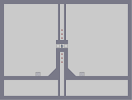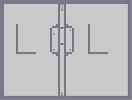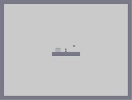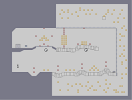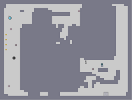simple yet hard III simple yet hard IV STRANGE BUG but where's the other half? my autogener4te Up and down

Pages: (0)

### well

this program dosnt seem to use z-snap so it seems a little pointless. and i dont rate anything that uses a program. (there is rarely exceptions - but they depend on the program) other than that - its alright

### sry

it's written in a fairly obscure computer language (HMPP)

### Its still pretty amazing

even though you used a program.
Btw, send the program my way if you get time.
-decemberunderground3@hotmail.com

program located?

### oh yeah

Do NOT try to load it, it's a waste of time